• 为了解决此限制，我们提出了一个新的分类器，称为随机子空间证据分类器（RSEC）。 具体而言，RSEC首先计算每个类的局部超平面距离，不仅作为整个特征空间的证据，而且还作为随机生成的特征子空间的证据。 然后，...
• 六、随机子空间 Random subspace method Random subspace method（RSM）又叫attribute bagging 或者 feature bagging，是集成学习的一种。随机子空间通过使用随机的部分特征而不是所有的特征来训练每个分类器，来...
六、随机子空间  Random subspace method
Random subspace method（RSM）又叫attribute bagging 或者 feature bagging，是集成学习的一种。随机子空间通过使用随机的部分特征而不是所有的特征来训练每个分类器，来降低每个分类器之间的相关性。
类似bagging, bagging是随机使用部分训练数据，而Random subspace method是随机使用部分特征，因此Random subspace method常用语特征远远大于训练样本数的情况，比如核磁共振、基因组序列、CSI（信道状态信息）。
实际上，随机森林就是一个使用了RSM和bagging的decision tree.同样的，RSM也可以用在SVM等其他分类器上。
在训练出每个分类器之后进行预测，得到每个分类器对应的结果。根据多数投票或结合先验概率的方法获得最终结果。

七、stacking
Stacking(有时候也称之为stacked generalization)是指训练一个模型用于组合(combine)其他各个模型。即首先先训练多个不同的模型，然后再以之前训练的各个模型的输出为输入来训练一个模型，以得到一个最终的输出。如果可以选用任意一个组合算法，那么理论上，Stacking可以表示各种Ensemble方法。然而，实际中，我们通常使用单层logistic回归作为组合模型。

先在整个训练数据集上通过bootstrapped抽样得到各个训练集合，得到一系列分类模型，称之为Tier 1分类器, 然后将输出用于训练Tier 2 分类器(meta-classifier, Wolpert 1992)。潜在的一个思想是希望训练数据都得被正确的学习到了。比如某个分类器错误的学习到了特征空间里某个特定区域，因此错误分类就会来自这个区域，但是Tier 2分类器可能根据其他的分类器学习到正确的分类。交叉验证也通常用于训练Tier 1分类器：把这个训练集合分成T个块，Tier 1中的每个分类器根据各自余下的T-1块进行训练，并在T块（该块数据并未用于训练）上测试。之后将这些分类器的输出作为输入，在整个训练集合上训练Tier 2分类器。（这里未提及测试集，测试集是指不在任何训练过程中出现的数据）。

七、总结与回顾

回顾集成学习有效的第二个前提，怎样形成不同的基本分类器呢？主要从以下5个方面得到。
1.基本分类器本身的种类，即其构成算法不同。如SVM, decision tree等。
2.对数据进行处理不同，比如说boosting, bagging, stacking, cross-validation, hold-outtest等。
3.对输入特征进行处理和选择，如RSM
4.对输出结果进行处理，比如说有的学者提出的纠错码
5.引入随机扰动。在每个基本分类器的学习过程之中引入随机扰动，使得学习出来的每个基本分类器都不同，这是用于进行人工神经网络集成学习的最常见方法。

下面是一些实验性的结论：
Boosting方法的集成分类器效果明显优于bagging,但是在某些数据集boosting算法的效果还不如单个分类器的。
使用随机化的人工神经网络初始权值来进行集成的方法往往能够取得和bagging同样好的效果。
Boosting算法一定程度上依赖而数据集，而bagging对数据集的依赖没有那么明显。
Boosting算法不仅能够减少偏差还能减少方差，但bagging算法只能减少方差，对偏差的减少作用不大。

部分转载自：
http://www.datakit.cn/blog/2014/11/02/Ensemble_learning.html
http://blog.csdn.net/wangle1e/article/details/51955856?locationNum=1&fps=1
http://blog.csdn.net/u011345885/article/details/52797077?locationNum=7&fps=1
有删改。

参考：
清华大学廖英毅， "集成学习综述."  soft.cs.tsinghua.edu.cn/~keltin/docs/ensemble.pdf.
展开全文集成学习
• 本文就对集成学习中Bagging与随机森林算法做一个总结。 　随机森林是集成学习中可以和梯度提升树GBDT分庭抗礼的算法，尤其是它可以很方便的并行训练，在如今大数据大样本的的时代很有诱惑力。...
前言：在集成学习算法中，我们讲到了集成学习主要有两个流派，一个是boosting流派，它的特点是各个弱学习器之间有依赖关系。另一种是bagging流派，它的特点是各个弱学习器之间没有依赖关系，可以并行拟合。本文就对集成学习中Bagging与随机森林算法做一个总结。
随机森林是集成学习中可以和梯度提升树GBDT分庭抗礼的算法，尤其是它可以很方便的并行训练，在如今大数据大样本的的时代很有诱惑力。

1、bagging的原理
Bagging：基于数据随机重抽样的分类器构建方法。从训练集从进行子抽样组成每个基模型所需要的子训练集，对所有基模型预测的结果进行综合产生最终的预测结果。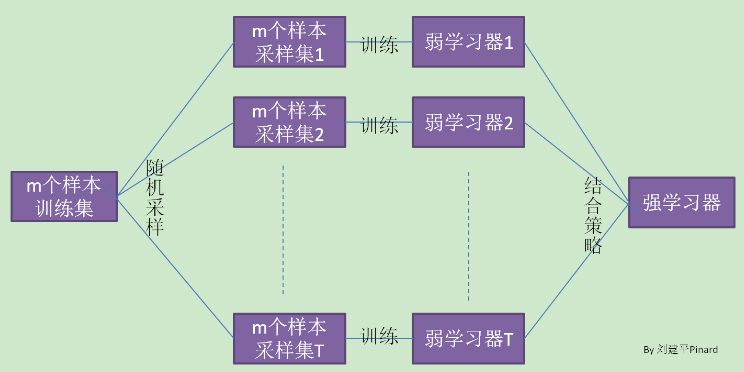它的特点在“随机采样”。那么什么是随机采样？
随机采样(bootsrap)就是从我们的训练集里面采集固定个数的样本，但是每采集一个样本后，都将样本放回。也就是说，之前采集到的样本在放回后有可能继续被采集到。对于我们的Bagging算法，一般会随机采集和训练集样本数m一样个数的样本。这样得到的采样集和训练集样本的个数相同，但是样本内容不同。如果我们对有m个样本训练集做T次的随机采样，，则由于随机性，T个采样集各不相同。
注意到这和GBDT的子采样是不同的。GBDT的子采样是无放回采样，而Bagging的子采样是放回采样。

2、随机森林算法
2.1基本原理
理解了bagging算法，随机森林(Random Forest,以下简称RF)就好理解了。它是Bagging算法的进化版，也就是说，它的思想仍然是bagging,但是进行了独有的改进。
RF引入了bagging和随机子空间的思想。随机森林是一个用算法构建的没有剪枝的分类决策树的集合，森林的输出釆用简单多数投票法，或者是单棵树输出结果的简单平均得到。
具体的训练过程如下：
步骤1 利用bagging思想，随机产生样本子集。步骤2 利用随机子空间思想，随机抽取f个特征，进行节点分裂，构建单棵回归决策子树。步骤3 重复步骤1、步骤2，构建T棵回归决策子树，每棵树自由生长，不进行剪枝，形成森林。步骤4 T棵决策子树的预测值取平均，作为最终结果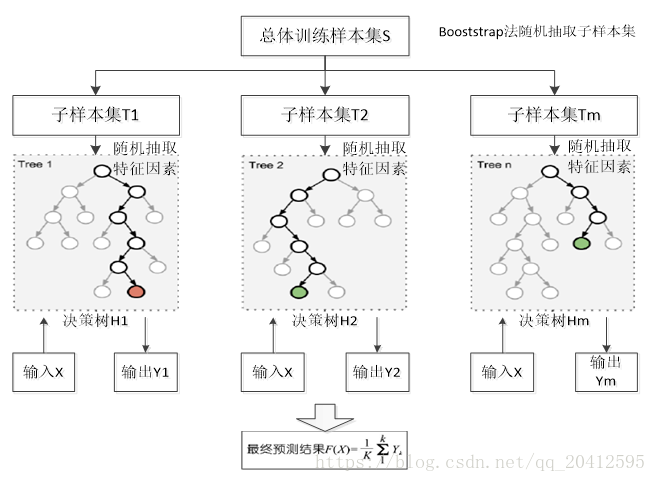随机：数据采样随机，特征选择随机森林：很多个决策树并行放在一起（决策树的详细讲解）
首先，RF使用了CART决策树作为弱学习器，这让我们想到了梯度提升树GBDT。第二，在使用决策树的基础上，RF对决策树的建立做了改进，对于普通的决策树，我们会在节点上所有的n个样本特征中选择一个最优的特征来做决策树的左右子树划分，但是RF通过随机选择节点上的一部分样本特征，这个数字小于n，假设为，然后在这些随机选择的个样本特征中，选择一个最优的特征来做决策树的左右子树划分。这样进一步增强了模型的泛化能力。
如果=n，则此时RF的CART决策树和普通的CART决策树没有区别。越小，则模型越健壮，当然此时对于训练集的拟合程度会变差。也就是说越小，模型的方差会减小，但是偏差会增大。在实际案例中，一般会通过交叉验证调参获取一个合适的的值。
除了上面两点，RF和普通的bagging算法没有什么不同， 下面简单总结下RF的算法。
2.2、随机森林的加深理解
1）对随机森林采样的理解
对于一个样本，它在某一次含m个样本的训练集的随机采样中，每次被采集到的概率是。不被采集到的概率为。如果m次采样都没有被采集中的概率是。当时，。也就是说，在bagging的每轮随机采样中，训练集中大约有36.8%的数据没有被采样集采集中。
对于这部分大约36.8%的没有被采样到的数据，我们常常称之为袋外数据(out of bag, 简称oob)。这些数据没有参与训练集模型的拟合，因此可以用来检测模型的泛化能力。
袋外错误率（oob error，out-of-bag error）: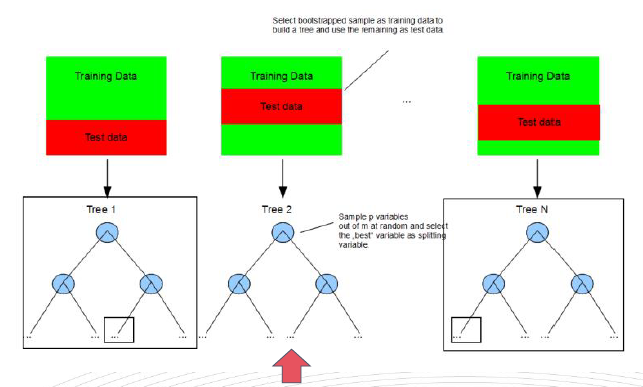对“袋外”样本进行多棵决策树的融合预测，得到的预测值与真实值的误差率就是“袋外错误率”oob有了oob，我们发现随机森林算法不需要单独的测试集来防止过拟合，oob就可以作为单独测试集的作用
3、随机森林算法参数
class sklearn.ensemble.RandomForestClassifier(n_estimators=10, criterion=’gini’, max_depth=None, min_samples_split=2, min_samples_leaf=1, min_weight_fraction_leaf=0.0, max_features=’auto’, max_leaf_nodes=None, min_impurity_decrease=0.0, min_impurity_split=None, bootstrap=True, oob_score=False, n_jobs=1, random_state=None, verbose=0, warm_start=False, class_weight=None)[source]¶（随机森林分类参数官网）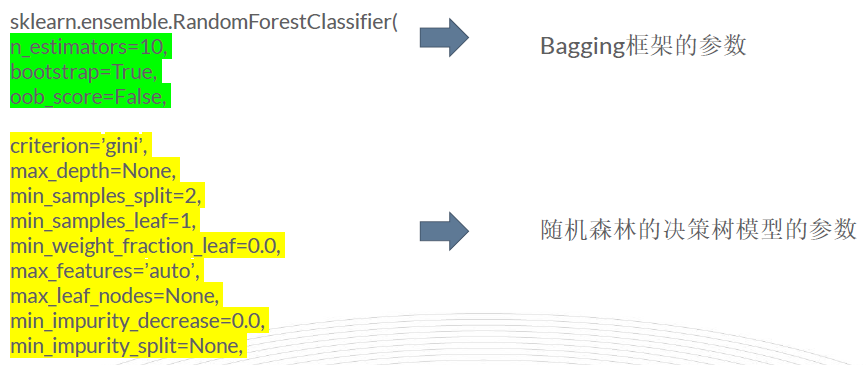对集成算法而言，有集成模型的框架参数，也有基模型的框架参数，我们要分清其中的意义。
首先我们关注于RF的Bagging框架的参数
n_estimators: 也就是弱学习器的最大迭代次数，或者说最大的弱学习器的个数。一般来说n_estimators太小，容易欠拟合，n_estimators太大，计算量会太大，并且n_estimators到一定的数量后，再增大n_estimators获得的模型提升会很小，所以一般选择一个适中的数值。默认是100。oob_score :即是否采用袋外样本来评估模型的好坏。默认是False。推荐设置为True，因为袋外分数反应了一个模型拟合后的泛化能力。criterion: 即CART树做划分时对特征的评价标准。分类模型和回归模型的损失函数是不一样的。分类RF对应的CART分类树默认是基尼系数gini,另一个可选择的标准是信息增益。回归RF对应的CART回归树默认是均方差mse，另一个可以选择的标准是绝对值差mae。一般来说选择默认的标准就已经很好的。
RF的决策树参数
决策树的框架参数这里就不做阐述。决策树的讲解部分有讲。
模型的输出结果Attributes
Attributes: estimators_ : list of DecisionTreeClassifier

The collection of fitted sub-estimators.
classes_ : array of shape = [n_classes] or a list of such arrays

The classes labels (single output problem), or a list of arrays of class labels (multi-output problem).
n_classes_ : int or list

The number of classes (single output problem), or a list containing the number of classes for each output (multi-output problem).
n_features_ : int

The number of features when fit is performed.
n_outputs_ : int

The number of outputs when fit is performed.
feature_importances_ : array of shape = [n_features]

The feature importances (the higher, the more important the feature).
oob_score_ : float

Score of the training dataset obtained using an out-of-bag estimate.
oob_decision_function_ : array of shape = [n_samples, n_classes]

Decision function computed with out-of-bag estimate on the training set. If n_estimators is small it might be possible that a data point was never left out during the bootstrap. In this case, oob_decision_function_ might contain NaN.

常用的可以用到特征重要性，可以作为特征选择的一个方法。
4、随机森林算法小结
最后也提一下，随机森林有很多的变种，感兴趣的可以自己研究。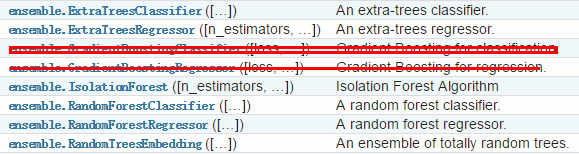优点：
预测效果比较好训练可以高度并行化，对于大数据时代的大样本训练速度有优势。能处理高维特征，不需要提前做特征过滤不容易过拟合模型训练速度快（相比Boosting），容易做并行化处理能对特征的重要性排序
缺点：
对少量数据集和低维数据集的分类不一定可以得到很好的效果执行速度虽然比Boosting等快，但还是比单个的决策树慢很多

参考资料：
https://blog.csdn.net/julialove102123/article/details/78405284
https://www.cnblogs.com/pinard/p/6156009.html
展开全文• 随机森林（Random forest，简称RF）是由Leo Breiman在...总的来说，随机森林就是在随机子空间中随机组合的自由生长的CART决策树+Bagging得到的。 这里在CART决策树前加入了三个描述词，一个是在随机子空间中，也...
如果只想大致理解下随机森林的原理请看第一部分，第二部分是对原论文的研读（灰色引用标记的为证明或自己的理解），这部分可能需要花的时间比较长，不需要的可以忽略。 此外，文末列出来的参考视频，如果读论文还是不太懂个人觉得很有必要看看，会加深理解，因为读完论文再看了一遍也让我对之前脑海中的袋外估计、特征重要性等内容有了推掉重来的印象。
第一部分：理解
随机森林（Random forest，简称RF）是由Leo Breiman在2001年在《Machine Learning》（IF:2.809，2018）正式发表提出。正如上一篇博客中写的，随机森林属于集成学习中Bagging的典型算法。总的来说，随机森林就是在随机子空间中随机组合的自由生长的CART决策树+Bagging得到的。
这里在CART决策树前加入了三个描述词，一个是在随机子空间中，也就是指数据随机采样，即随机地在样本空间中选取样本子空间；一个是随机组合，也就是特征随机采样，将一些特征随机组合在一起，可以只是选出来的这些特征进行学习，也可以将某些特征进行组合成新的特征进行学习，总之都在这个随机的子空间中；还有一个自由生长，这个比较好理解，就是对每个决策树都不进行剪枝。
对于随机森林的理解可以分为“随机”和“森林”：“随机”是指数据随机采样以及特征随机采样，主要体现在Bagging，它的全称是Bootstrap aggregation，称为自助法，它是一种有放回的抽样方法。Bagging的每个弱学习器的训练集都是通过随机且有放回采样得到的，简单来说就是每次从原始样本中随机且有放回的采样n个训练样本，进行T轮采样，得到T个训练集，然后用这T个训练集，分别独立训练T个弱学习器，这T个弱学习器结合成一个强学习器，强学习器的结果作为整体的结果输出。而“森林”则是指利用多颗自由生长的CART决策树组成一个“森林”，作为上述的T个弱学习器。
随机森林的生成规则如下：
从原始训练集中随机有放回采样n个训练样本，重复T次；用这T个数据集，分别作为训练集训练T个CART树模型；如果特征维度为M，指定一个常数m（网上说一般小于等于

M

\sqrt{M}

，论文中尝试了

i

n

t

(

l

o

g

2

M

+

1

)

int(log_2M+1)

），随机地从M个特征中选取m个特征子集，每次树进行分裂时，从这m个特征中选择最优的；将生成的T棵决策树组成随机森林，保证每棵树都尽最大程度的生长，并且没有剪枝过程；对于分类问题，由T个CART树投票表决产生分类结果。对于回归问题，由T棵树预测结果的均值作为最终的预测结果。
由于数据采样的随机性，每棵树的训练集都是不同的，而且里面包含重复的训练样本，对于一个含有异常值的数据来说，这种采样方式可以令大多数的树模型得到不包含或者只包含少量异常点的数据，再经过多个学习器最终的“投票”或者“平均”，可以使得随机森林对异常值不敏感，有很强的抗干扰能力。而特征随机采样可以说是随机森林自带的特征选择的功能，正如前面所说，实际数据中会有很多多余甚至无关特征，这些特征会严重影响模型效果，随机森林中让每棵决策树只选择少量的特征进行学习，最后再合成他们的学习成果，因此随机森林能处理高维数据，并且不用做特征选择。
Out of Bag
此外，Bagging中还涉及到一个比较重要的概念：OOB（Out of Bag），意思是，通过统计，有放回的抽样，有一部分样本会多次出现，而另一部分样本不出现。Bagging每轮随机采样中，假如说训练集很大，而且抽的次数很多，也就是

n

n

很大，那训练集中大约有

(

1

−

1

n

)

n

=

1

(

n

n

−

1

)

n

=

1

(

1

+

1

n

−

1

)

n

→

1

e

≈

36.8

%

(1-{1\over n})^n= {1\over ({n\over n-1})^n}= {1\over (1+{1\over n-1})^n}\to {1\over e} \thickapprox 36.8\%

的数据没有被采样集采集。对于这部分大约

1

3

1\over3

没有被采样的数据，称为袋外数据。这些数据没有参与模型的训练，可以用来测试模型的泛化能力，所以随机森林可以不需要单独留出交叉验证的验证集。下图是机器学习技法中的ppt，描述了随机森林的袋外误差是怎样计算的，具体为：
对于数据集中每一个样本

(

x

i

,

y

i

)

(x_i, y_i)

，将所有未使用该样本

(

x

i

,

y

i

)

(x_i, y_i)

进行训练得到的决策树组成的子随机森林模型

G

i

−

G_i^-

；对

G

i

−

G_i^-

进行误差计算，即得到样本

(

x

i

,

y

i

)

(x_i, y_i)

进行交叉验证的结果，看看这个子模型在样本

(

x

i

,

y

i

)

(x_i, y_i)

的表现；（此步骤与使用留一法类似）重复步骤2，依次计算在剩余每个样本；对所有样本进行交叉验证的结果进行求和取平均，作为随机森林模型最终的交叉验证的结果。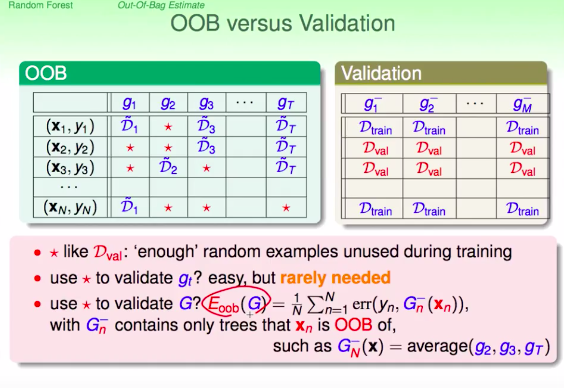特征重要性：
如果某个特征对于这个模型很重要，那将这个特征用一些噪音替换之后的表现将会下降，而替换前后的差值就可以用来选择重要的特征，因为特征越重要对模型的表现影响越大，因此差值也越大。 但是若替换成一些噪音，例如高斯或者均匀分布的噪音，会影响训练集原本的分布，因此采用permutation，这里的大概意思就是将数据重新洗牌。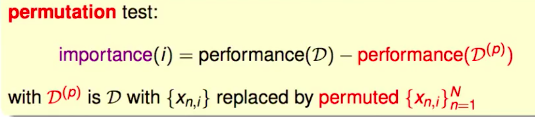而原始的洗牌需要在训练数据上做的，并且每次洗牌后需要重新训练一个模型，再进行验证得出模型的performance，而随机森林的表现由上述所说就是袋外误差

E

O

O

B

(

G

)

E_{OOB}(G)

来评估，但是随机森林不需要在训练的时候做洗牌，而是在验证的时候洗牌，就是直接使用OOB的那部分数据上来替换掉需要测试重要性的特征的袋外误差，然后这样计算出每一个特征的重要性。总的说来随机森林的特征重要性是由permutation+OOB得到。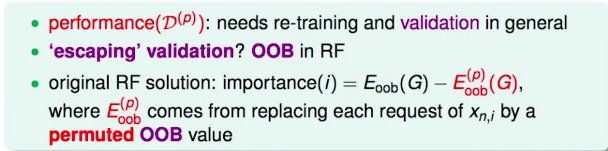这样的优点是不需要在原始数据上做洗牌，因而也不需要重新训练模型，在训练完一个随机森林的模型后，即可使用该模型来计算特征重要性。
注： 目前在Python的机器学习工具scikit-learn中随机森林的特征重要性不是上述这样做，而是通过（树中的决策节点的）特征使用的相对顺序（即深度）来进行评估。 决策树顶部使用的特征对更大一部分输入样本的最终预测决策做出贡献；因此，可以使用接受每个特征对最终预测的贡献的样本比例来评估该 特征的相对重要性 。 通过对多个随机树中的 预期贡献率 （expected activity rates） 取平均，可以减少这种估计的方差 ，并将其用于特征选择。 详见scikit-learn (sklearn) 官方文档
随机森林的优点：
由于每棵树之间是没有相关性的，因此可以并行生成，使得整个算法运行十分高效；自由生长的决策树很容易过拟合，但是由于Bagging使得随机森林对异常值不敏感，有很强的抗干扰能力；CART决策树有很好的性质，例如可以处理缺失值、处理连续或者多分类的变量等；可以用来做特征选择。
第二部分：论文研读
主要内容框架（不含介绍）：
Breiman L. Random Forests[J]. Machine Learning, 2001, 45(1):5-32. 这篇文章主要是受到Amit和Geman在1997发表的Shape quantization and recognition with randomized trees一文的启发。他们从大量几何定义的特征中随机选择一些浅层树来定义每个节点上的分割，从而生成手写字符识别的浅层树。尽管随机森林的实现是不同的，也不是基于特定问题的，但他们的工作为随机森林的想法提供了开端。
对于第

k

k

个决策树，就有一个随机向量

Θ

k

\Theta_k

，它与之前的随机向量

Θ

1

,

…

,

Θ

k

−

1

\Theta_1,\dots,\Theta_{k-1}

独立但是同分布；这样一个由训练集和

Θ

k

\Theta_k

长成的树就对应了一个分类器

h

(

x

,

Θ

k

)

h(x,\Theta_k)

，其中

x

x

是输入向量。由此给出随机森林的定义，即：
定义1.1 随机森林是一个树状结构分类器组成的分类器集合

{

h

(

x

,

Θ

k

)

,

k

=

1

,

…
&ThinSpace;

}

\{h(x,\Theta_k),k=1,\dots\}

，其中

{

Θ

k

}

\{\Theta_k\}

是独立同分布的随机向量，并且每棵树在输入

x

x

下得到一个类别由此投票选出最有可能的类别。
随机森林的收敛
给定一组分类器

h

1

(

x

)

,

h

2

(

x

)

,

…

,

h

K

(

x

)

h_1(x),h_2(x),\dots,h_K(x)

，它的的训练集是从随机向量

Y

,

X

Y,X

的分布中随机抽取的，定义margin函数为

m

g

(

X

,

Y

)

=

a

v

k

I

(

h

k

(

X

)

=

Y

)

−

max

⁡

j

≠

Y

a

v

k

I

(

h

k

(

X

)

=

j

)

mg(X,Y)=av_kI(h_k(X)=Y)-\max_{j\neq Y}av_kI(h_k(X)=j)

其中

I

(

⋅

)

I(\cdot)

为示性函数。则这个margin函数描述了在

Y

,

X

Y,X

上分类正确的平均投票数超过分类为其他任何类别的平均投票数的程度，它类似于回归问题中的残差

y

−

f

(

x

)

y-f(x)

，但不同的是，这个margin越大，分类就越可信。 给定泛化误差为

P

E

∗

=

P

X

,

Y

(

m

g

(

X

,

Y

)

&lt;

0

)

PE^*=P_{X,Y}(mg(X,Y)&lt;0)

这里对于泛化误差我的理解有以下两种： 1、因为泛化误差的定义为：学到的模型

f

~

\tilde{f}

对未知数据预测的误差，即

R

e

x

p

(

f

~

)

=

E

p

[

L

(

Y

,

f

~

(

X

)

)

]

R_{exp}(\tilde{f})=E_p[L(Y,\tilde{f}(X))]

，其中

L

(

⋅

)

L(\cdot)

为损失函数。这里的margin函数相当于损失函数，因为margin越大，分类就越可信，因此假定分布为伯努利分布，即

m

g

&lt;

0

mg&lt;0

时取1，

m

g

&gt;

0

mg&gt;0

时取0，则

E

p

[

L

(

Y

,

f

~

(

X

)

)

]

=

1

×

P

X

,

Y

(

m

g

(

X

,

Y

)

&lt;

0

)

+

0

×

P

X

,

Y

(

m

g

(

X

,

Y

)

&gt;

0

)

=

P

E

∗

E_p[L(Y,\tilde{f}(X))]=1\times P_{X,Y}(mg(X,Y)&lt;0)+0\times P_{X,Y}(mg(X,Y)&gt;0)=PE^*

2、分类任务中错误率的定义为：

E

(

f

,

D

)

=

1

m

∑

i

=

1

m

I

(

f

(

x

i

)

≠

y

i

)

→

P

(

I

(

f

(

x

i

)

≠

y

i

)

E(f,D)={1\over m}\sum_{i=1}^mI(f(x_i)\neq y_i)\to P(I(f(x_i)\neq y_i)

，类似的，对于margin来说我们评价分类错误超过分类正确的情况，这样定义一个性能度量

P

E

∗

=

P

X

,

Y

(

m

g

(

X

,

Y

)

&lt;

0

)

PE^*=P_{X,Y}(mg(X,Y)&lt;0)

来评价泛化误差也是很自然的。

在随机森林中

h

k

(

X

)

=

h

(

x

,

Θ

k

)

h_k(X)=h(x,\Theta_k)

，因此，由强大数定律就有：
定理1.2 随着树的数量增加，对于序列

Θ

1

,

Θ

2

,

…

\Theta_1,\Theta_2,\dots

，泛化误差

P

E

∗

PE^*

几乎处处收敛到

P

X

,

Y

(

P

Θ

(

h

(

X

,

Θ

)

=

Y

)

−

max

⁡

j

≠

Y

P

Θ

(

h

(

X

,

Θ

)

=

j

)

&lt;

0

)

P_{X,Y}(P_\Theta(h(X,\Theta)=Y)-\max_{j\neq Y}P_\Theta(h(X,\Theta)=j)&lt;0)

证明：只需证明在序列空间上

Θ

1

,

Θ

2

,

…

\Theta_1,\Theta_2,\dots

上存在一个零测集

C

C

，使得对所有

x

∉

C

x\notin C

，有

1

N

∑

n

=

1

N

I

(

h

(

Θ

n

,

x

)

=

j

)

→

P

Θ

(

h

(

Θ

n

,

x

)

=

j

)

{1\over N}\sum_{n=1}^NI(h(\Theta_n,x)=j)\to P_\Theta(h(\Theta_n,x)=j)

对固定的训练集和固定的

Θ

\Theta

，使得

h

(

Θ

,

x

)

=

j

h(\Theta,x)=j

成立的所有

x

x

是一个hyper-rectangle的并，对所有

h

(

Θ

,

x

)

h(\Theta,x)

，只存在唯一的有限

K

K

个这样的hyper-rectangle的并，表示为

S

1

,

…

,

S

K

S_1,\dots,S_K

。若

{

x

:

h

(

Θ

,

x

)

=

j

}

=

S

k

\{x:h(\Theta,x)=j\}=S_k

，定义

φ

(

Θ

)

=

k

\varphi(\Theta)=k

。令

N

k

N_k

表示为前

N

N

次实验中

φ

(

Θ

)

=

k

\varphi(\Theta)=k

成立的次数。则有

1

N

∑

n

=

1

N

I

(

h

(

Θ

n

,

x

)

=

j

)

=

1

N

∑

k

N

k

I

(

x

∈

S

k

)

{1\over N}\sum_{n=1}^NI(h(\Theta_n,x)=j)= {1\over N}\sum_{k}N_kI(x\in S_k)

由大数定律，有

N

k

=

1

N

∑

n

=

1

N

I

(

φ

(

Θ

n

)

=

k

)

→

a

.

s

.

P

Θ

(

φ

(

Θ

n

)

=

k

)

N_k={1\over N}\sum_{n=1}^NI(\varphi(\Theta_n)=k) \xrightarrow{a.s.}P_\Theta(\varphi(\Theta_n)=k)

对于某些不收敛的

k

k

，取所有集合的并，得到零测集

C

C

，使得在

C

C

外面，有

1

N

∑

n

=

1

N

I

(

h

(

Θ

n

,

x

)

=

j

)

→

∑

k

P

Θ

(

φ

(

Θ

n

)

=

k

)

I

(

x

∈

S

k

)

=

P

Θ

(

h

(

Θ

n

,

x

)

=

j

)

{1\over N}\sum_{n=1}^NI(h(\Theta_n,x)=j) \to \sum_{k}P_{\Theta}(\varphi(\Theta_n)=k)I(x\in S_k)=P_\Theta(h(\Theta_n,x)=j)

这个定理解释了为什么随机森林不会因为树的增加而过拟合，反而产生了一个泛化误差的有界值。
强度和相关系数（泛化误差的上界）
对于随机森林，可以用两个参数来表示泛化误差的上界，这两个参数是衡量单个分类器的精确度以及它们之间的相关性的指标。
定义2.1 随机森林的margin函数为

m

r

(

X

,

Y

)

=

P

Θ

(

h

(

X

,

Θ

)

=

Y

)

−

max

⁡

j

≠

Y

P

Θ

(

h

(

X

,

Θ

)

=

j

)

mr(X,Y)=P_\Theta(h(X,\Theta)=Y)-\max_{j\neq Y}P_\Theta(h(X,\Theta)=j)

分类器集合

{

h

(

x

,

Θ

)

}

\{h(x,\Theta)\}

的强度为

s

=

E

X

,

Y

m

r

(

X

,

Y

)

s=E_{X,Y}mr(X,Y)

假设

s

&gt;

0

s&gt;0

，由切比雪夫不等式有

(4)

P

E

∗

⩽

v

a

r

(

m

r

)

/

s

2

PE^*\leqslant var(mr)/s^2\tag{4}

因为

P

E

∗

=

P

X

,

Y

(

m

r

(

X

,

Y

)

&lt;

0

)

⩽

P

X

,

Y

(

m

r

&lt;

0

)

+

P

X

,

Y

(

m

r

&gt;

2

s

)

=

P

X

,

Y

(

∣

m

r

−

s

∣

&gt;

s

)

⩽

v

a

r

(

m

r

)

/

s

2

PE^*=P_{X,Y}(mr(X,Y)&lt;0)\leqslant P_{X,Y}(mr&lt;0)+P_{X,Y}(mr&gt;2s)=P_{X,Y}(|mr-s|&gt;s) \leqslant var(mr)/s^2

令

j

^

(

X

,

Y

)

=

arg

⁡

max

⁡

j

≠

Y

P

Θ

(

h

(

X

,

Θ

)

=

j

)

\hat{j}(X,Y)=\arg\max_{j\neq Y}P_\Theta(h(X,\Theta)=j)

，则

m

r

(

X

,

Y

)

=

P

Θ

(

h

(

X

,

Θ

)

=

Y

)

−

P

Θ

(

h

(

X

,

Θ

)

=

j

^

(

X

,

Y

)

)

=

E

Θ

[

I

(

h

(

X

,

Θ

)

=

Y

)

−

I

(

h

(

X

,

Θ

)

=

j

^

(

X

,

Y

)

)

]

\begin{aligned} mr(X,Y)&amp;=P_\Theta(h(X,\Theta)=Y)-P_\Theta(h(X,\Theta)=\hat{j}(X,Y))\\ &amp;=E_\Theta[I(h(X,\Theta)=Y)-I(h(X,\Theta)=\hat{j}(X,Y))] \end{aligned}

定义2.2 raw margin函数定义为

r

m

g

(

Θ

,

X

,

Y

)

=

I

(

h

(

X

,

Θ

)

=

Y

)

−

I

(

h

(

X

,

Θ

)

=

j

^

(

X

,

Y

)

)

rmg(\Theta,X,Y)=I(h(X,\Theta)=Y)-I(h(X,\Theta)=\hat{j}(X,Y))

则

m

r

(

X

,

Y

)

mr(X,Y)

是

r

m

g

(

Θ

,

X

,

Y

)

rmg(\Theta,X,Y)

关于

Θ

\Theta

取期望。 对任意的函数

f

f

，当

Θ

,

Θ

′

\Theta,\Theta&#x27;

相互独立且同分布时等式

[

E

Θ

f

(

Θ

)

]

2

=

E

Θ

,

Θ

′

f

(

Θ

)

f

(

Θ

′

)

[E_{\Theta}f(\Theta)]^2=E_{\Theta,\Theta&#x27;}f(\Theta)f(\Theta&#x27;)

恒成立。所以有

m

r

(

X

,

Y

)

2

=

E

Θ

,

Θ

′

r

m

g

(

Θ

,

X

,

Y

)

r

m

g

(

Θ

′

,

X

,

Y

)

mr(X,Y)^2=E_{\Theta,\Theta&#x27;} rmg(\Theta,X,Y)rmg(\Theta&#x27;,X,Y)

因此，

v

a

r

(

m

r

)

=

E

Θ

,

Θ

′

(

c

o

v

X

,

Y

r

m

g

(

Θ

,

X

,

Y

)

r

m

g

(

Θ

′

,

X

,

Y

)

)

=

E

Θ

,

Θ

′

(

ρ

(

Θ

,

Θ

′

)

s

d

(

Θ

)

s

d

(

Θ

′

)

)

\begin{aligned} var(mr)&amp;=E_{\Theta,\Theta&#x27;} (cov_{X,Y}rmg(\Theta,X,Y)rmg(\Theta&#x27;,X,Y))\\ &amp;=E_{\Theta,\Theta&#x27;}(\rho(\Theta,\Theta&#x27;)sd(\Theta)sd(\Theta&#x27;)) \end{aligned}

其中

ρ

(

Θ

,

Θ

′

)

\rho(\Theta,\Theta&#x27;)

是固定

Θ

,

Θ

′

\Theta,\Theta&#x27;

时

r

m

g

(

Θ

,

X

,

Y

)

rmg(\Theta,X,Y)

与

r

m

g

(

Θ

′

,

X

,

Y

)

rmg(\Theta&#x27;,X,Y)

之间的相关系数，

s

d

(

Θ

)

sd(\Theta)

是固定

Θ

\Theta

时

r

m

g

(

Θ

,

X

,

Y

)

rmg(\Theta,X,Y)

的标准差。则

(7)

v

a

r

(

m

r

)

=

ρ

ˉ

(

E

Θ

s

d

(

Θ

)

)

2

⩽

ρ

ˉ

E

Θ

v

a

r

(

Θ

)

(

v

a

r

(

s

d

)

=

E

s

d

2

−

(

E

s

d

)

2

⩾

0

)

\begin{aligned} var(mr)&amp;=\bar{\rho}(E_\Theta sd(\Theta))^2\\ &amp;\leqslant \bar{\rho}E_\Theta var(\Theta)\ \ \ (var(sd)=Esd^2-(Esd)^2\geqslant 0) \end{aligned}\tag{7}

其中

ρ

ˉ

\bar{\rho}

是相关系数的均值，即

ρ

ˉ

=

E

Θ

,

Θ

′

(

ρ

(

Θ

,

Θ

′

)

s

d

(

Θ

)

s

d

(

Θ

′

)

)

/

E

Θ

,

Θ

′

(

s

d

(

Θ

)

s

d

(

Θ

′

)

)

\bar{\rho}=E_{\Theta,\Theta&#x27;}(\rho(\Theta,\Theta&#x27;)sd(\Theta)sd(\Theta&#x27;))/E_{\Theta,\Theta&#x27;}(sd(\Theta)sd(\Theta&#x27;))

。而

(8)

E

Θ

v

a

r

(

Θ

)

⩽

E

Θ

(

E

X

,

Y

r

m

g

(

Θ

,

X

,

Y

)

)

2

−

s

2

⩽

1

−

s

2

\begin{aligned} E_\Theta var(\Theta)&amp;\leqslant E_\Theta(E_{X,Y}rmg(\Theta,X,Y))^2-s^2\\ &amp;\leqslant 1-s^2 \end{aligned} \tag{8}

E

Θ

v

a

r

(

Θ

)

⩽

v

a

r

Θ

(

E

(

Θ

)

)

?

=

v

a

r

Θ

(

E

X

,

Y

r

m

g

(

Θ

,

X

,

Y

)

)

=

E

Θ

(

E

X

,

Y

r

m

g

(

Θ

,

X

,

Y

)

)

2

−

(

E

Θ

E

X

,

Y

r

m

g

(

Θ

,

X

,

Y

)

)

2

=

E

Θ

(

E

X

,

Y

r

m

g

(

Θ

,

X

,

Y

)

)

2

−

(

E

X

,

Y

E

Θ

r

m

g

(

Θ

,

X

,

Y

)

)

2

=

E

Θ

(

E

X

,

Y

r

m

g

(

Θ

,

X

,

Y

)

)

2

−

(

E

X

,

Y

m

r

(

X

,

Y

)

)

2

=

E

Θ

(

E

X

,

Y

r

m

g

(

Θ

,

X

,

Y

)

)

2

−

s

2

⩽

1

−

s

2

\begin{aligned} E_\Theta var(\Theta)&amp;\leqslant var_\Theta(E(\Theta))?\\ &amp;= var_\Theta(E_{X,Y}rmg(\Theta,X,Y))\\ &amp;= E_\Theta(E_{X,Y}rmg(\Theta,X,Y))^2-(E_{\Theta}E_{X,Y}rmg(\Theta,X,Y))^2\\ &amp;= E_\Theta(E_{X,Y}rmg(\Theta,X,Y))^2-(E_{X,Y}E_{\Theta}rmg(\Theta,X,Y))^2\\ &amp;= E_\Theta(E_{X,Y}rmg(\Theta,X,Y))^2-(E_{X,Y}mr(X,Y))^2\\ &amp;= E_\Theta(E_{X,Y}rmg(\Theta,X,Y))^2-s^2\\ &amp;\leqslant 1-s^2 \end{aligned}

由式

(

4

)

,

(

7

)

,

(

8

)

(4),(7),(8)

得到下面定理：
定理2.3 泛化误差的上界为

P

E

∗

⩽

ρ

ˉ

(

1

−

s

2

)

/

s

2

PE^*\leqslant \bar{\rho}(1-s^2)/s^2

虽然这个界可能很松，但它对随机森林的提示功能与VC类型上界对其他类型分类器的提示功能相同。结果表明，随机森林泛化误差涉及的两个因素是森林中单个分类器的强度，以及它们之间关于raw margin函数的相关系数。c/s2比值是相关系数除以强度的平方。对于理解随机森林的作用，这个比值将是一个有效的指导——越小越好。

这里的

s

s

就是前面说所得类似于分类器精度的一个性能度量，

ρ

\rho

是关于raw margin函数的相关系数，因此上述推导说明泛化误差的上界只与分类器精度以及分类器之间的某种相关系数有关。

定义2.4 随机森林的c/s2比值定义为

c

/

s

2

=

ρ

ˉ

/

s

2

c/s2=\bar{\rho}/s^2

在二分类情形下，margin函数为

m

r

(

X

,

Y

)

=

2

P

Θ

(

h

(

X

,

Θ

)

=

Y

)

−

1

mr(X,Y)=2P_\Theta(h(X,\Theta)=Y)-1

强度为正的要求(见(4))与我们熟悉的弱学习条件

E

X

.

Y

P

Θ

(

h

(

X

,

Θ

)

=

Y

)

&gt;

0.5

E_{X.Y}P_\Theta(h(X,\Theta)=Y)&gt;0.5

相似。（即弱学习器的准确度要比随便猜好一些）。raw margin函数为

2

I

(

h

(

X

,

Θ

)

=

Y

)

−

1

2I(h(X,\Theta)=Y)-1

，

ρ

ˉ

\bar{\rho}

是

I

(

h

(

X

,

Θ

)

=

Y

)

I(h(X,\Theta)=Y)

与

I

(

h

(

X

,

Θ

′

)

=

Y

)

I(h(X,\Theta&#x27;)=Y)

之间的相关系数。特别地，若

Y

Y

的值取

+

1

+1

和

−

1

-1

，则

ρ

ˉ

=

E

Θ

,

Θ

′

[

ρ

(

h

(

⋅

,

Θ

)

,

h

(

⋅

,

Θ

′

)

)

]

\bar{\rho}=E_{\Theta,\Theta&#x27;}[\rho(h(\cdot,\Theta),h(\cdot,\Theta&#x27;))]

因此

ρ

ˉ

\bar{\rho}

是在平均分布

Θ

,

Θ

′

\Theta,\Theta&#x27;

下森林中两颗不同的决策树之间的相关系数。 对于多分类任务，之前定义的强度既取决于森林，又取决于单独的每棵树，因为是森林决定了

j

^

(

X

,

Y

)

\hat{j}(X,Y)

。另外，因为

P

E

∗

=

P

X

,

Y

(

P

Θ

(

h

(

X

,

Θ

)

=

Y

)

−

max

⁡

j

≠

Y

P

Θ

(

h

(

X

,

Θ

)

=

j

)

&lt;

0

)

⩽

∑

j

P

X

,

Y

(

P

Θ

(

h

(

X

,

Θ

)

=

Y

)

−

P

Θ

(

h

(

X

,

Θ

)

=

j

)

&lt;

0

)

\begin{aligned} PE^*&amp;=P_{X,Y}(P_\Theta(h(X,\Theta)=Y)-\max_{j\neq Y}P_\Theta(h(X,\Theta)=j)&lt;0)\\ &amp;\leqslant \sum_j P_{X,Y}(P_\Theta(h(X,\Theta)=Y)-P_\Theta(h(X,\Theta)=j)&lt;0) \end{aligned}

令

s

j

=

E

X

,

Y

(

P

Θ

(

h

(

X

,

Θ

)

=

Y

)

−

P

Θ

(

h

(

X

,

Θ

)

=

j

)

)

s_j=E_{X,Y}(P_\Theta(h(X,\Theta)=Y)-P_\Theta(h(X,\Theta)=j))

表示分类器

h

(

x

,

Θ

k

)

h(x,\Theta_k)

相对于第

j

j

类的强度。注意强度的定义不依赖于森林。由切比雪夫不等式，假设所有的

s

j

&gt;

0

s_j&gt;0

，则有

P

E

∗

⩽

∑

j

v

a

r

(

P

Θ

(

h

(

X

,

Θ

)

=

Y

)

−

P

Θ

(

h

(

X

,

Θ

)

=

j

)

)

s

j

2

PE^* \leqslant \sum_j var(P_\Theta(h(X,\Theta)=Y)-P_\Theta(h(X,\Theta)=j))s_j^2

类似于

(

7

)

(7)

式得推导，这个方差也可以写成平均相关系数。本文并没有估计上式的经验值。
随机森林的优点
为了提高精度,随机性必须最小化相关系数

ρ

ˉ

\bar{\rho}

袋外估计
在随机森林实验中，bagging与随机特征选择相结合。每一个新的训练集都从原始的训练集中有放回的抽取出来，然后在新的训练集上使用随机特征选择生成一棵树，长出来的树不修剪。
使用bagging有两个原因。首先，当使用随机特征时，使用bagging似乎可以提高准确性。第二，bagging可用于对组合树的泛化误差(PE)进行持续估计，以及对强度和相关系数的估计。
假设有一种从任意训练集构造分类器的方法，给定一个特定的训练集

T

T

，形成boostrap训练集

T

k

T_k

，构造分类器

h

(

x

,

T

k

)

h(x, T_k)

，让它们投票组成一个包装好的预测器。对于训练集中的每个

y

,

x

y,x

，只对那些不包含

y

,

x

y,x

的分类器

T

k

T_k

进行汇总，称之为袋外分类器。这样对泛化误差的袋外估计为训练集上袋外分类器的错误率。

也就是之前机器学习技法视频中给出的那个计算公式，对泛化误差的袋外估计为

E

O

O

B

(

G

)

E_{OOB}(G)

，

G

n

−

(

x

n

)

G_n^-(x_n)

就是这里所说的袋外分类器，它的错误率就是所有袋外分类器的平均值，因此

E

O

O

B

(

G

)

=

1

N

∑

n

=

1

N

e

r

r

(

y

n

,

G

n

−

(

x

n

)

)

E_{OOB}(G)={1\over N}\sum_{n=1}^N err(y_n,G_n^-(x_n))

。

在每个boostrap训练集中，约有三分之一的样本被遗漏。因此，袋外估计是基于组合的分类器数量仅为当前主组合的三分之一左右。由于错误率随着组合数量的增加而降低，袋外估计往往会高估当前的错误率。为了得到无偏的袋外估计，有必要运行超过测试集错误收敛的点。但与交叉验证不同的是，交叉验证存在偏差但其程度未知，而袋外估计是无偏的。
强度和相关系数也可以用袋外法估计。这给出了有助于理解分类精度以及如何提高分类精度的内部估计。
随机输入选择
最简单的具有随机特征的随机森林是通过随机选择在每个节点上用来做划分的一小组输入变量而形成的。使用CART方法生成树，且最大化树的形状，不要剪枝，用Forest-RI表示这个过程，组的大小

F

F

是固定的。这里试了两个

F

F

的值。第一个只使用一个随机选择的变量，即

F

=

1

F = 1

。第二个

F

F

是第一个小于

l

o

g

2

M

+

1

log_2 M+1

的整数，其中

M

M

是输入的个数。
作者的实验使用了来自UCI存储库的13个较小的数据集、3个较大的数据集，这些数据集被分成训练和测试集以及4个合成数据集。表1给出了一个简要的总结（这里不列出来）。
在这13个较小的数据集中，每一个都使用以下步骤：随机留出10%的数据。在剩下的数据上，随机森林运行两次，第一次

F

=

1

F = 1

，第二次

F

=

i

n

t

(

l

o

g

2

M

+

1

)

F = int(log_2 M + 1)

表2中第二列是通过最小的袋外误差从两组规模中选取的结果。第三列是只使用一个随机特性来生成树的测试集误差。第四列包含了为最佳设置(单个或选择)计算的森林中单个树的泛化误差的袋外估计（单颗树的平均误差）。这个估计值是通过在每棵生长的树中使用遗漏的实例作为测试集，并对森林中所有树的结果求平均值来计算的。

F

F

值而不是预先设置的两个值，这种比较可能会更有利。但该方法对

F

F

值不太敏感，

F

=

1

F = 1

时的错误率与

F

F

值较高时的错误率的平均绝对差小于1%。这种差异在三个大数据集中表现得最为明显。
之所以包括单变量测试集的结果，是因为在一些数据集中，使用一个随机输入变量比使用多个更好。在其他组中，结果仅略好于使用单一变量。令人惊讶的是，在每个节点上使用一个随机选择的输入变量进行分割可以产生良好的精度。（表2中明显可以看出）

F

∗

l

o

g

2

(

N

)

/

M

F∗log_2(N)/M

，其中

F

F

是Forest-RI中使用的变量的数量，

N

N

是样本的数量，

M

M

是输入变量的数量。对于邮政编码数据，使用

F

=

1

F = 1

，这个比率是0.025，这意味着Forest-RI要快40倍。实证检验证实了这一差异。在250Mhz的Macintosh上，对邮政编码数据执行Forest-RI (

F

=

1

F = 1

输入的线性组合
如果只有少量的输入，比如

M

M

，将

F

F

取

M

M

中相当大的一部分可能会导致强度的增加，但相关系数也更高。另一种方法是通过对一些输入变量进行随机线性组合来定义更多的特征。也就是说，通过指定要组合的变量数

L

L

来生成一个特性。在给定的节点上，随机选取

L

L

个变量，并将其与

[

−

1

,

1

]

[- 1,1]

上的均匀随机数相加。生成

F

F

个线性组合，然后在这些组合上搜索最佳分割。这个过程称为Forest-RC。
我们使用

L

=

3

L=3

和

F

=

2

,

8

F= 2,8

，

F

F

的选择由袋外估计决定。我们选择

L

=

3

L =3

，是因为对于

O

(

M

3

)

O(M^3)

不同的输入变量组合，

F

F

的值越大，在增加强度的同时，相关系数不会有太大的上升。如果输入变量在一个数据集是不能比较的，他们需要做标准化处理。测试集的结果在表3给出，第三列包含

F

=

2

F = 2

的结果。第四列包含表2所计算的单个树的结果。
除了三个较大的数据集外，

F

=

8

F = 8

的使用非常没必要；

F

=

2

F = 2

接近最小值。对于较大的数据集，

F

=

8

F = 8

在表2和表3中有一些项，其中所选的项小于一个输入值或Forest-RC小于两个特征的值。发生这种情况的原因是，当与

F

F

的两个值对应的错误率接近时，out-of-bag估计将几乎随机地选择

F

F

的值。
进行了一项小型调查，以确定是否可以改进这三个较大数据集的性能。根据第6节卫星数据的运行情况，我们推测强度在较大的数据集中不断上升，而相关系数更快地达到渐近线。因此，我们对较大的数据集分别使用100、100和200棵树进行了

F

=

100

F = 100

的运行。在卫星数据上，误差降至8.5%，在字母数据上，误差降至3.0%，但压缩码测试集误差没有减小。根据一种有根据的预感，我尝试了

F

=

25

F = 25

的Forest-RI。邮政编码测试集错误降低到5.8%。这是迄今为止树集成在这三个数据集上实现的最低测试集错误。
分类变量
一些或所有的输入变量可能是分类变量，因为我们想定义变量的加法组合，所以我们需要定义如何处理分类变量，以便它们可以与数值变量组合。作者的方法是，每次在一个节点上选择一个分类变量进行分割时，选择该变量类别的一个随机子集，并定义一个替代变量，当该变量的分类值在子集中且在子集外为零时，该替代变量为1。
由于具有

I

I

值的分类变量可以编码为

I

−

1

I - 1

虚拟的0 - 1变量，所以我们使变量

I

−

1

I - 1

的概率是数值变量的1倍。当许多变量是分类变量时，使用

F

F

的低值会导致低相关系数，但也会导致低强度。

F

F

必须增加到大约2 - 3倍

i

n

t

(

l

o

g

2

M

+

1

)

int(log_2 M + 1)

，以获得足够的强度来提供良好的测试集精度。
例如，在有60个四值分类的DNA数据集上，训练集中有2000个样本，测试集中有1186个样本，使用

F

=

20

F = 20

F

=

12

F = 12

这种方法的一个优点是，它克服了如何处理具有许多值的分类的困难。在二分类问题中，这可以通过使用Breiman等人(1985)提出的机制来避免，该机制将对最佳分类分割的搜索简化为

O

(

I

)

O(I)

计算。对于更多的类，搜索最佳分类分割是一个

O

(

2

I

−

1

)

O(2^{I - 1})

计算。在随机森林实现中，对任何类别变量的计算只涉及类别的随机子集的选择。
强度与相关系数的实证结果
为了对各种数据集中强度和相关系数的影响进行实证研究，使用了强度和相关系数的out-of-bag估计。
首先对声纳数据(60个输入，208个示例)使用1到50个输入运行Forest-RI。在每次迭代中，将10%的数据分割成一个测试集，然后将每个节点上随机选择的输入数

F

F

从1变化到50。对于每一个

F

F

值，生长100棵树形成一个随机森林，记录下测试集误差、强度、相关系数等的终值。进行了80次迭代，每次随机删除10%的数据作为测试集使用，所有结果的平均值都超过80次。在这个实验中总共生成了40万棵树。
图1的顶部图表绘制了强度和相关系数的值与

F

F

的关系。当

F

=

4

F = 4

时，强度保持不变，增加更多的输入没有帮助。但相关系数持续增加。第二个图绘制了测试集误差和对

F

F

的泛化误差的袋外估计。袋外估计更为稳定。两者都表现出相同的行为——从

F

=

1

F = 1

到

F

F

在4-8之间有一个小的下降，然后是一个普遍的、逐渐的上升。误差的增加与强度的稳定区域的开始相吻合。
图2显示了在breast数据集中类似的运行情况，其中使用了由三个输入的随机组合组成的特征。这些特征的数量从1个到25个不等。同样，相关系数显示出缓慢的上升，而强度实际上保持不变，因此最小误差是在F = 1处。令人惊讶的是，这两个数字的强度相对稳定。由于相关系数缓慢而稳定地增加，当只使用少量输入或特性时，误差最小。
由于较大的数据集似乎与较小的数据集具有不同的表现，所以作者对卫星数据集进行了类似的实验。特征的数量(每个特征由两个输入的随机加和组成)从1到25不等，每个特征集组合100个分类器。结果如图3所示。结果与较小数据集的结果不同。相关系数和强度均有小幅但稳定的增长。错误率略有下降。我们推测，随着更大、更复杂的数据集的出现，这种强度在稳定下来之前持续增长的时间更长。
作者的结果表明，较好的(较低的泛化误差)随机森林分类器之间的相关关系越低，分类器的强度越大。建树过程中使用的随机性主要为了低相关系数

ρ

ˉ

\bar{\rho}

所以随机森林之所以表现好，就是在保证了单个分类器的性能的同时，分类器之间相关性比较低。

可以修改各种分类器，使其同时使用训练集和训练集上的一组权重。考虑如下的随机森林：定义了训练集上

K

K

个不同的非负的和为一的权值集合，用

w

(

1

)

,

w

(

2

)

,

…

,

w

(

K

)

w(1),w(2),\dots,w(K)

表示。与这些权重对应的是概率

p

(

1

)

,

p

(

2

)

,

…

,

p

(

K

)

p(1),p(2),\dots,p(K)

，其和为1。根据这些概率，从

1

,

…

,

K

1,\dots,K

中抽取整数，结果即为

Θ

\Theta

。若

Θ

=

k

\Theta=k

， 则用训练集来生成一个带权重

w

(

k

)

w (k)

的分类器

h

(

x

,

Θ

)

h(x,\Theta)

。

w

(

1

)

,

w

(

2

)

,

…

,

w

(

50

)

w(1),w(2),\dots,w(50)

(前25个被丢弃)。第

k

k

组权值的概率与

Q

(

w

k

)

=

l

o

g

[

(

1

−

e

r

r

o

r

(

k

)

)

/

e

r

r

o

r

(

k

)

]

Q(w_k) = log[(1 - error(k))/error(k)]

成正比，其中

e

r

r

o

r

(

k

)

error(k)

为第

k

k

分类器的

w

(

k

)

w(k)

加权训练集误差。然后森林被运行250次。

w

(

k

+

1

)

=

ϕ

(

w

(

k

)

)

w (k + 1) =\phi(w (k))

，其中

ϕ

\phi

是由基分类器决定的函数。用

h

(

x

,

w

k

)

h(x,w_k)

表示第

k

k

个分类器。第k个分类器的投票由

Q

(

w

k

)

Q(w_k)

加权，因此第

j

j

类在

x

x

时的标准化投票为

(10)

∑

k

I

(

h

(

x

,

w

k

)

=

j

)

Q

(

w

k

)

/

∑

k

Q

(

w

k

)

\sum_kI(h(x,w_k)=j)Q(w_k)/\sum_kQ(w_k)\tag{10}

对于任何定义在权重空间上的函数，定义算子

T

f

(

w

)

=

f

(

ϕ

(

w

)

)

T f(w) = f(\phi(w))

。我们猜想，

T

T

是对于不变测度

π

(

d

w

)

\pi(dw)

是遍历的。则

(

10

)

(10)

式会以分布

Q

π

(

d

w

)

=

Q

(

w

)

π

(

d

w

)

/

∫

Q

(

v

)

π

(

d

v

)

Q\pi(dw) = Q (w)\pi(dw) / \int Q(v)\pi(dv)

收敛到

E

Q

π

[

I

(

h

(

x

,

w

)

=

j

)

]

E_{Q\pi}[I(h (x, w) = j)]

Q

π

Q\pi

中随机选择的。
输出噪声的影响
对于实验中的每一个数据集，随机抽取10%作为一个测试集，对剩余的训练集进行两次运行，第一次运行在训练集上。第二次运行是在训练集的噪声版本上。噪声版本是通过随机地将5%的类别标签更改为从其他标签中均匀地选择的替代类标签而得到的。
具有许多弱输入的数据
具有弱输入的数据集在医学诊断、文献检索等领域越来越普遍。其共同特征是没有单个输入或小组输入可以区分类别。这种类型的数据对于通常的分类器(神经网络和树)来说是困难的。
为了查看Forest-RI方法是否有可能工作，生成了10个类，1000个二元输入数据。文中代码生成了一组概率

{

p

(

j

,

m

)

}

\{p(j, m)\}

，其中

j

j

是类标签，

m

m

是输入号。然后，类

j

j

的输入是一个由

M

M

个二元变量组成的字符串，第M个变量以概率

p

(

j

,

M

)

p(j, M)

为1。
对于训练集，

N

=

1000

N = 1000

。还使用相同的

{

p

(

j

,

k

)

}

\{p (j, k)\}

构造了一个4000个样本的测试集。对代码的检查表明，每个类在某些位置具有更高的潜在概率。但是所有这些位置的总数大约是2000个，所以有很大的重叠。假设我们知道所有的

{

p

(

j

,

k

)

}

\{p (j, k)\}

，那么在我们的运行中计算的特定

{

p

(

j

,

m

)

}

\{p (j, m)\}

的贝叶斯错误率为1.0%。
由于输入之间是相互独立的，根据训练数据估计

{

p

(

j

,

k

)

}

\{p (j, k)\}

的朴素贝叶斯分类器应该是最优的，而且错误率为6.2%。这并不是对朴素贝叶斯的认可，因为很容易在输入之间建立依赖关系，从而增加朴素贝叶斯的错误率。作者用这个例子是因为贝叶斯错误率和朴素贝叶斯错误率很容易计算。
作者从

F

=

1

F = 1

的Forest-RI开始。它收敛得非常慢，经过2500次迭代，当它停止时，仍然没有收敛。测试集误差为10.7%。强度为0.069，c/s2比值为2.5，相关系数为0.012。尽管强度很低，但几乎为零的相关系数意味着，随着迭代的进行，我们在增加小的精度增量。
显然，我们想要的是在保持低相关系数的同时增加强度。再次使用

F

=

i

n

t

(

l

o

g

2

M

+

1

)

=

10

F = int(log_2 M + 1) = 10

运行Forest-RI。结果令人鼓舞。经过2000次迭代，它收敛了。测试集误差为3.0%。强度为0.22，相关系数为0.045，c/s2为0.91。按照这个趋势，Forest-RI以

F

=

25

F = 25

运行，迭代2000次后停止。测试集误差为2.8%。强度为0.28，相关性为0.065，c/s2为0.83。
有趣的是，Forest-RI可以产生比贝叶斯错误率高不了多少的错误率。单个分类器是弱分类器。

F

=

1

F = 1

时，平均树错误率为80%，

F

=

10

F = 10

时，是65%，对于

F

=

25

F = 25

探索随机森林机制（特征的重要性）
就对其机制的简单解释而言，由树组成的森林是很费解的。在一些应用中，例如医学实验分析中，理解变量之间的相互作用是至关重要的，它提供了预测的准确性。首先使用内部的out-of-bag估计，并通过仅使用选定的变量重新运行来验证。
假设有

M

M

个输入变量。在构建每棵树之后，袋外样本中的第

m

m

个变量的值被随机排列，袋外数据运行到相应的树中。对每个袋外的

x

n

x_n

给出的分类将被保存，从

m

=

1

,

2

,

…

,

M

m = 1,2,\dots,M

重复，在运行结束时，将关于第

m

m

个混噪音变量的

x

n

x_n

的多数袋外类别投票与

x

n

x_n

的真实类标签进行比较，给出一个误分类率。
输出是与袋外率(所有变量不变)相比，误分类率增加的百分比。我们通过使用一个包含1000棵树且没有测试集的森林的单次运行来得到这些估计。
在糖尿病数据集中，仅使用

F

=

1

F = 1

的单变量，由于变量的噪声，误差的上升如图4所示。
第二个变量是迄今为止最重要的，其次是变量8和变量6。在100次重复中只使用变量2运行随机森林，每次只留下10%来测量测试集的误差，得到的误差为29.7%，而使用所有变量时的误差为23.1%。但是当变量8被添加时，误差只下降到29.4%。当变量6与变量2相加时，误差降至26.4%。
变量6看起来很重要，但一旦输入变量2就没有帮助了，这是因变量如何影响随机森林预测误差的一个特性。假设有两个变量

x

1

x_1

和

x

2

x_2

是相同的，并且具有重要的预测信息。因为在随机森林中，每一个都是以相同的频率被选中的，所以分别对每一个进行噪声处理将导致相同的错误率增加。但是，一旦

x

1

x_1

作为预测变量，再使用

x

2

x_2

将不会带来任何错误率的下降。在糖尿病数据集中，第8个变量与第2个变量携带一些相同的信息。因此，当与第二个变量结合使用时，它不会增加预测精度。
相对于所使用的输入特性，错误率上升的相对幅度相当稳定。上面的实验是用

F

=

2

F = 2

的三个输入的组合重复进行的。结果如图5所示。
另一个有趣的例子是投票数据。这有435个例子对应于435个国会议员和16个变量反映他们对16个问题的赞成-反对投票。分类变量是共和党人还是民主党人。为了了解哪些问题是最重要的，我们再次运行生成1000棵树的噪音变量程序。使用

F

=

5

F = 5

的单个输入获得原始数据的最低错误率，因此在运行中使用了这些参数。图6中的结果。
变量4很突出，如果变量4有噪声，则错误为三倍。我们仅使用变量4重新运行这个数据集。测试集误差为4.3%，与使用所有变量时的误差基本相同。4票的表决结果把共和党人和民主党人区分开来，就像4票的表决结果以及其他15个议题的表决结果组合一样。
本节给出的方法只是一个开始。需要更多的研究来理解如何给出一个更完整的图景。
随机森林回归
随机森林回归是由依赖于一个随机向量?

Θ

\Theta

的生成树组成，使得这样树预测

h

(

x

,

Θ

)

h(x,\Theta)

为数值，而不是分类标签。输出值为数值，并且假设训练集独立从随机向量

Y

,

X

Y, X

的分布中抽样。任意数值预测器

h

(

x

)

h(x)

的均方泛化误差为

E

X

,

Y

(

Y

−

h

(

X

)

)

2

E_{X,Y}(Y-h(X))^2

随机森林预测器由决策树

{

h

(

x

,

Θ

)

}

\{h(x,\Theta)\}

对

k

k

取平均得到。
定理11.1 随着森林中树的数量趋于无穷

(12)

E

X

,

Y

(

Y

−

a

v

k

h

(

X

,

Θ

)

)

2

→

a

.

s

.

E

X

,

Y

(

Y

−

E

Θ

h

(

X

,

Θ

)

)

2

E_{X,Y}(Y-av_kh(X,\Theta))^2\xrightarrow{a.s.}E_{X,Y}(Y-E_\Theta h(X,\Theta))^2\tag{12}

将

(

12

)

(12)

式右边项记为

P

E

∗

(

f

o

r

e

s

t

)

PE^*(forest)

——随机森林的泛化误差。将决策树的平均泛化误差定义为：

P

E

∗

(

t

r

e

e

)

=

E

Θ

E

X

,

Y

(

Y

−

h

(

X

,

Θ

)

)

2

PE^*(tree)=E_\Theta E_{X,Y}(Y-h(X,\Theta))^2

定理11.2 假设对所有的

Θ

\Theta

，

E

Y

=

E

X

h

(

X

,

Θ

)

EY=E_Xh(X,\Theta)

，则

P

E

∗

(

f

o

r

e

s

t

)

⩽

ρ

ˉ

P

E

∗

(

t

r

e

e

)

PE^*(forest)\leqslant \bar{\rho}PE^*(tree)

其中

ρ

ˉ

\bar{\rho}

是残差项

Y

−

h

(

X

,

Θ

)

Y-h(X,\Theta)

和

Y

−

h

(

X

,

Θ

′

)

Y-h(X,\Theta&#x27;)

之前的加权相关系数，

Θ

,

Θ

′

\Theta,\Theta&#x27;

相互独立。

证明：

P

E

∗

(

f

o

r

e

s

t

)

=

E

X

,

Y

[

E

Θ

(

Y

−

h

(

X

,

Θ

)

)

]

2

=

E

Θ

E

Θ

′

E

X

,

Y

(

Y

−

h

(

X

,

Θ

)

)

(

Y

−

h

(

X

,

Θ

′

)

)

\begin{aligned} PE^*(forest)&amp;=E_{X,Y}[E_\Theta (Y-h(X,\Theta))]^2\\ &amp;=E_\Theta E_{\Theta&#x27;} E_{X,Y} (Y-h(X,\Theta))(Y-h(X,\Theta&#x27;)) \end{aligned}

等式右边为协方差，可以写为：

E

Θ

E

Θ

′

(

ρ

(

Θ

,

Θ

′

)

s

d

(

Θ

)

s

d

(

Θ

′

)

)

E_\Theta E_{\Theta&#x27;} (\rho(\Theta,\Theta&#x27;)sd(\Theta)sd(\Theta&#x27;))

，其中

s

d

(

Θ

)

=

E

X

,

Y

(

Y

−

h

(

X

,

Θ

)

)

2

sd(\Theta)=\sqrt{E_{X,Y} (Y-h(X,\Theta))^2}

，定义加权相关系数为：

ρ

ˉ

=

E

Θ

,

Θ

′

(

ρ

(

Θ

,

Θ

′

)

s

d

(

Θ

)

s

d

(

Θ

′

)

)

/

(

E

Θ

s

d

(

Θ

)

)

2

\bar{\rho}=E_{\Theta,\Theta&#x27;}(\rho(\Theta,\Theta&#x27;)sd(\Theta)sd(\Theta&#x27;))/(E_{\Theta}sd(\Theta))^2

则有：

P

E

∗

(

f

o

r

e

s

t

)

=

ρ

ˉ

(

E

Θ

s

d

(

Θ

)

)

2

⩽

ρ

ˉ

P

E

∗

(

t

r

e

e

)

PE^*(forest)=\bar{\rho}(E_\Theta sd(\Theta))^2\leqslant \bar{\rho}PE^*(tree)

定理(11.2)指出了精确回归森林的要求——残差之间的低相关系数以及低误差决策树。随机森林减少树木的平均误差受限于系数

ρ

ˉ

\bar{\rho}

。所采用的随机化需要针对低相关性。
回归的实证结果
在回归森林中，我们在bagging上使用随机特征选择。因此,我们可以使用由out-of-bag估计提供的监控给出

P

E

∗

(

f

o

r

e

s

t

)

,

P

E

∗

(

t

r

e

e

)

PE^*(forest),PE^*(tree)

和

ρ

ˉ

\bar{\rho}

的估计。这些估计数的推导与分类中的类似。整个过程中，使用由两个输入的随机线性加和组成的特征。稍后我们将讨论这些特性中有多少可以用于确定每个节点上的分割。使用的特征越多较

P

E

∗

(

t

r

e

e

)

PE^*(tree)

越低，但

ρ

ˉ

\bar{\rho}

会更高。在我们的实证研究中使用了以下数据集，见表5。
在这些数据集中，波士顿住房，Abalone和Servo可在UCI存储库获取。机械臂数据由迈克尔·乔丹提供。最后三个数据集是合成的。它们起源于Friedman (1991)， Breiman (1998b)也对它们进行了描述。这些数据集是用来比较适应性套袋和套袋的相同的数据集(见Breiman, 1999)，除了一个合成数据集(Peak20)被排除了，这个数据集被其他研究人员和作者自己都发现是异常的。
列出的前三个数据集大小适中，测试集误差的估计方法是去掉10%的随机实例，在剩下的90%上运行，并使用剩下的10%作为测试集。重复100次，测试集误差取平均值。鲍鱼数据集更大，有4,177个实例和8个输入变量。它最初有25%的实例作为测试集。我们运行这个数据集时，将随机选择的25%的实例作为测试集使用，重复10次并取平均值。
表6给出了bagging、自适应bagging和随机森林的测试集均方误差。这些都是使用25个特征运行的，每个特征都是两个随机选择的输入的随机线性组合，来分割每个节点，每个特征都是两个输入的随机组合。所有的方法都运行了所有数据集，结合100棵树。在所有数据集中，都强制执行了“如果节点大小小于5，则不拆分”规则。
回归和分类之间一个有趣的区别是，相关系数随着使用的特征数量的增加而增长得非常慢。主要的影响是

P

E

∗

(

t

r

e

e

)

PE^*(tree)

的减少。因此，需要相对大量的特性来减少

P

E

∗

(

t

r

e

e

)

PE^*(tree)

并获得最佳的测试集误差近似值。
表6中的结果是混合的。随机森林-随机特征总是比bagging好。在自适应bagging使误差急剧减少的数据集中，森林所产生的误差减少并不明显。在自适应bagging并没有比bagging更好的数据集中，森林产生了改进。
对于相同数量的输入组合，在较宽的范围内，误差不会随着特性的数量发生太大的变化。如果使用的数量过小，

P

E

∗

(

t

r

e

e

)

PE^*(tree)

就会变得过大，误差就会增加。如果使用的数量太大，相关系数就会上升，误差也会增加。介于两者之间的范围通常很大。在这个范围内，随着特性数量的增加，相关系数会增加，但是

P

E

∗

(

t

r

e

e

)

PE^*(tree)

会通过减少来进行补偿。
表7给出了测试集误差、袋外误差估计和对

P

E

∗

(

t

r

e

e

)

PE^*(tree)

的袋外估计以及相关系数。
正如预期的那样，OB的误差估计一直很高。机器人手臂的数据比较低，但作者认为这是单独的训练集和测试集造成的伪影，其中测试集的错误率可能略高于训练集。
作为一个实验，作者没有使用bagging，并将其替换为随机输出(Breiman, 1998b)。在这个过程中，每个输出都加入了均值为零的高斯噪声。噪声的标准差等于输出的标准差。与bagging实验类似，树的构建使用了25个特征，每个特征由两个随机选择的输入随机线性组合而成，用于分割每个节点。结果如表8所示。
前两个数据集的错误率是迄今为止最低的。总的来说，添加输出噪声与随机特征选择比bagging效果更好。这说明了随机森林设置的灵活性——可以添加各种随机组合来查看哪种方法效果最好。
评价和结论
随机森林是一种有效的预测工具。由于大数定律，它们不会过拟合。加入正确的随机性使它们成为精确的分类器和回归器。此外，根据单个预测器的强度和它们之间的相关系数，该框架可以洞察随机森林的预测能力。采用袋外估计，使强度和相关系数的理论值具体化。
在一段时间内，传统的想法是森林不能与arcing类型的算法在精确性方面竞争。我们的研究结果消除了这种想法，但也引出了一些有趣的问题。boosting和arcing算法具有减少偏差和方差的能力(Schapire et al.，1998)。回归中的自适应bagging算法(Breiman, 1999)是为了减少偏差而设计的，在分类和回归中都能有效地运行。但是，就像arcing一样，它也会随着训练的进展而改变训练集。
森林提供了与boosting和自适应bagging相比较的结果，但并没有显著地改变训练集。它们的精度表明它们的作用是减少偏差。其机制并不明显。随机森林也可以看作是贝叶斯预测。虽然作者怀疑这是一个富有成效的探索路线，如果它可以解释偏差减少，作者可能更偏向于贝叶斯。
随机输入和随机特征在分类上有很好的效果，而在回归分析中效果较差。本研究中唯一使用的随机性类型是bagging和随机特征。很有可能加入其他类型的随机性会给出更好的结果。例如，一位评审建议使用特征的随机布尔组合。
一个几乎显而易见的问题是，是否可以通过用结合随机特征和boosting来提高精度。对于较大的数据集，似乎可以显著降低错误率。在一些运行中，我们得到的错误低至5.1%的邮编数据，2.2%的字母数据和7.9%的卫星数据。在较小的数据集上，改进较少。这方面还需要做更多的工作，但它确实表明，加入不同的随机性可以产生更好的结果。
最近的一篇论文(Breiman, 2000)表明在二分类问题的分布空间中，随机森林等价于一个作用于真实margin的核。讨论了随机性(低相关系数)增强了核的对称性，而强度增强了在突变曲线边界处的理想偏度。希望这能说明相关性和强度的双重作用。Kleinberg(2000)关于随机区分的理论框架也有助于理解。

下一篇可以看看Breiman, L. 2000. Some infinity theory for predictor ensembles. Technical Report 579, Statistics Dept. UCB.

参考资料
Breiman L. Random Forests[J]. Machine Learning, 2001, 45(1):5-32. 机器学习技法（林轩田）
展开全文• 集成学习（ensemble learning）是目前非常流行的机器学习策略，基本上所有问题都可以借用其思想来得到效果上的提升。基本出发点就是把算法和各种策略集中在一起，说白了就是一个搞不定大家一起上！集成学习既可以...
集成算法
集成学习（ensemble learning）是目前非常流行的机器学习策略，基本上所有问题都可以借用其思想来得到效果上的提升。基本出发点就是把算法和各种策略集中在一起，说白了就是一个搞不定大家一起上！集成学习既可以用于分类问题，也可以用于回归问题，在机器学习领域会经常看到它的身影，本章就来探讨一下几种经典的集成策略，并结合其应用进行通俗解读。
Bagging算法
集成算法有3个核心的思想：bagging、boosting和stacking，这几种集成策略还是非常好理解的，下面向大家逐一介绍。
并行的集成
Bagging即boostrap aggregating，其中boostrap是一种有放回的抽样方法，抽样策略是简单的随机抽样。其原理很直接，把多个基础模型放到一起，最后再求平均值即可，这里可以把决策树当作基础模型，其实基本上所有集成策略都是以树模型为基础的，公式如下：

f

(

x

)

=

1

M

∑

m

=

1

M

f

m

(

x

)

f(x)=\frac{1}{M} \sum_{m=1}^{M} f_{m}(x)

首先对数据集进行随机采样，分别训练多个树模型，最终将其结果整合在一起即可，思想还是非常容易理解的，其中最具代表性的算法就是随机森林。
随机森林
随机森林是机器学习中十分常用的算法，也是bagging集成策略中最实用的算法之一。那么随机和森林分别是什么意思呢？森林应该比较好理解，分别建立了多个决策树，把它们放到一起不就是森林吗？这些决策树都是为了解决同一任务建立的，最终的目标也都是一致的，最后将其结果来平均即可，如图8-1所示。想要得到多个决策树模型并不难，只需要多次建模就可以。但是，需要考虑一个问题，如果每一个树模型都相同，那么最终平均的结果也相同。为了使得最终的结果能够更好，通常希望每一个树模型都是有个性的，整个森林才能呈现出多样性，这样再求它们的平均，结果应当更稳定有效。
如何才能保证多样性呢？如果输入的数据是固定的，模型的参数也是固定的，那么，得到的结果就是唯一的，如何解决这个问题呢？此时就需要随机森林中的另一部分—随机。这个随机一般叫作二重随机性，因为要随机两种方案，下面分别进行介绍。
首先是数据采样的随机，训练数据取自整个数据集中的一部分，如果每一个树模型的输入数据都是不同的，例如随机抽取80%的数据样本当作第一棵树的输入数据，再随机抽取80%的样本数据当作第二棵树的输入数据，并且还是有放回的采样，这就保证两棵树的输入是不同的，既然输入数据不同，得到的结果必然也会有所差异，这是第一重随机。
如果只在数据层面上做文章，那么多样性肯定不够，还需考虑一下特征，如果对不同的树模型选择不同的特征，结果的差异就会更大。例如，对第一棵树随机选择所有特征中的60%来建模，第二棵再随机选择其中60%的特征来建模，这样就把差异放大了，这就是第二重随机。如上图所示，由于二重随机性使得创建出来的多个树模型各不相同，即便是同样的任务目标，在各自的结果上也会出现一定的差异，随机森林的目的就是要通过大量的基础树模型找到最稳定可靠的结果，如下图所示，最终的预测结果由全部树模型共同决定。解释随机森林的概念之后，再把它们组合起来总结如下：
1.随机森林首先是一种并联的思想，同时创建多个树模型，它们之间是不会有任何影响的，使用相同参数，只是输入不同。2.为了满足多样性的要求，需要对数据集进行随机采样，其中包括样本随机采样与特征随机采样，目的是让每一棵树都有个性。3.将所有的树模型组合在一起。在分类任务中，求众数就是最终的分类结果；在回归任务中，直接求平均值即可。
对随机森林来说，还需讨论一些细节问题，例如树的个数是越多越好吗？树越多代表整体的能力越强，但是，如果建立太多的树模型，会导致整体效率有所下降，还需考虑时间成本。在实际问题中，树模型的个数一般取100～200个，继续增加下去，效果也不会发生明显改变。
下图是随机森林中树模型个数对结果的影响，可以发现，随着树模型个数的增加，在初始阶段，准确率上升很明显，但是随着树模型个数的继续增加，准确率逐渐趋于稳定，并开始上下浮动。这都是正常现象，因为在构建决策树的时候，它们都是相互独立的，很难保证把每一棵树都加起来之后会比原来的整体更好。当树模型个数达到一定数值后，整体效果趋于稳定，所以树模型个数也不用特别多，够用即可。在集成算法中，还有一个很实用的参数——特征重要性，如下图所示。先不用管每一个特征是什么，特征重要性就是在数据中每一个特征的重要程度，也就是在树模型中，哪些特征被利用得更多，因为树模型会优先选择最优价值的特征。在集成算法中，会综合考虑所有树模型，如果一个特征在大部分基础树模型中都被使用并且靠近根节点，它自然比较重要。当使用树模型时，可以非常清晰地得到整个分裂过程，方便进行可视化分析，如图8-6所示，这也是其他算法望尘莫及的，在后面的实战任务中将展示绘制树模型的可视化结果的过程。最后再来总结一下bagging集成策略的特点：
1.并联形式，可以快速地得到各个基础模型，它们之间不会相互干扰，但是其中也存在问题，不能确保加进来的每一个基础树模型都对结果产生促进作用，可能有个别树模型反而拉后腿。  2.可以进行可视化展示，树模型本身就具有这个优势，每一个树模型都具有实际意义。  3.相当于半自动进行特征选择，总是会先用最好的特征，这在特征工程中一定程度上省时省力，适用于较高维度的数据，并且还可以进行特征重要性评估。
Boosting算法
上一节介绍的bagging思想是，先并行训练一堆基础树模型，然后求平均。这就出现了一个问题：如果每一个树模型都比较弱，整体平均完还是很弱，那么怎样才能使模型的整体战斗力更强呢？这回轮到boosting算法登场了，boosting算法可以说是目前比较厉害的一种策略。
串行的集成
Boosting算法的核心思想就在于要使得整体的效果越来越好，整体队伍是非常优秀的，一般效果的树模型想加入进来是不行的，只要最强的树模型。怎么才能做到呢？先来看一下boosting算法的基本公式：

F

n

(

x

)

=

F

m

−

1

(

x

)

+

argmin

⁡

h

∑

i

=

1

n

L

(

y

i

,

F

m

−

1

(

x

i

)

+

h

(

x

i

)

)

F_{n}(x)=F_{m-1}(x)+\operatorname{argmin}_{h} \sum_{i=1}^{n} L\left(y_{i}, F_{m-1}\left(x_{i}\right)+h\left(x_{i}\right)\right)

通俗的解释就是把

F

m

−

1

(

x

)

F_{m−1}(x)

当作前一轮得到的整体，这个整体中可能已经包含多个树模型，当再往这个整体中加入一个树模型的时候，需要满足一个条件——新加入的

h

(

x

i

)

h(x_{i})

与前一轮的整体组合完之后，效果要比之前好。怎么评估这个好坏呢？就是看整体模型的损失是不是有所下降。
Boosting算法是一种串联方式，如下图所示，先有第一个树模型，然后不断往里加入一个个新的树模型，但是有一个前提，就是新加入的树模型要使得其与之前的整体组合完之后效果更好，说明要求更严格。最终的结果与bagging也有明显的区别，这里不需要再取平均值，而是直接把所有树模型的结果加在一起。那么，为什么这么做呢？回到银行贷款的任务中，假设数据的真实值等于1000，首先对树A进行预测，得到值950，看起来还不错。接下来树B登场了，这时出现一个关键点，就是它在预测的时候，并不是要继续预测银行可能贷款多少，而是想办法弥补树A还有多少没做好，也就是1000−950=50，可以把50当作残差，这就是树B要预测的结果，假设得到30。现在需要把树A和树B组合成为一个整体，它们一起预测得950+30=980。接下来树C要完成的就是剩下的残差（也就是20），那么最终的结果就是树A、B、C各自的结果加在一起得950+30+18=998，如下图所示。说到这里，相信大家已经有点感觉了，boosting算法好像开挂了，为了达到目标不择手段！没错，这就是boosting算法的基本出发点。下面再来介绍一下boosting算法中的一个典型代表——Adaboost算法。简单来说，Adaboost算法还是按照boosting算法的思想，要建立多个基础模型，一个个地串联在一起。
下图是Adaboost算法的建模流程。当得到第一个基础树模型之后，在数据集上有些样本分正确，有些样本分错误。此时需要考虑这样一个问题，为什么会分错呢？是不是因为这些样本比较难以判断吗？那么更应当注重这些难度较大的，也就是需要给样本不同的权重，做对的样本，权重相对较低，因为已经做得很好，不需要太多额外的关注；做错的样本权重就要增大，让模型能更重视它。以此类推，每一次划分数据集时，都会出现不同的错误样本，继续重新调整权重，以对数据集不断进行划分即可。每一次划分都相当于得到一个基础的树模型，它们要的目标就是优先解决之前还没有划分正确的样本。下图是Adaboost算法需要把之前的基础模型都串在一起得到最终结果，但是这里引入了系数，相当于每一个基础模型的重要程度，因为不同的基础模型都会得到其各自的评估结果，例如准确率，在把它们串在一起的时候，也不能同等对待，效果好的让它多发挥作用，效果一般的，让它参与一下即可，这就是系数的作用。Adaboost算法整体计算流程如下图所示，在训练每一个基础树模型时，需要调整数据集中每个样本的权重分布，由于每次的训练数据都会发生改变，这就使得每次训练的结果也会有所不同，最终再把所有的结果累加在一起。Stacking模型
前面讨论了bagging和boosting算法，它们都是用相同的基础模型进行不同方式的组合，而stacking模型与它们不同，它可以使用多个不同算法模型一起完成一个任务，先来看看它的整体流程，如下图所示。
首先选择m个不同分类器分别对数据进行建模，这些分类器可以是各种机器学习算法，例如树模型、逻辑回归、支持向量机、神经网络等，各种算法分别得到各自的结果，这可以当作第一阶段。再把各算法的结果（例如得到了4种算法的分类结果，二分类中就是0/1值）当作数据特征传入第二阶段的总分类器中，此处只需选择一个分类器即可，得到最终结果。
其实就是把无论多少维的特征数据传入各种算法模型中，例如有4个算法模型，得到的结果组合在一起就可以当作一个4维结果，再将其传入到第二阶段中得到最终的结果。下图是stacking策略的计算细节，其中Model1可以当作是第一阶段中的一个算法，它与交叉验证原理相似，先将数据分成多份，然后各自得到一个预测结果。那么为什么这么做呢？直接拿原始数据进行训练不可以吗？其实在机器学习中，一直都遵循一个原则，就是不希望训练集对接下来任何测试过程产生影响。在第二阶段中，需要把上一步得到的结果当作特征再进行建模，以得到最终结果，如果在第一阶段中直接使用全部训练集结果，相当于第二阶段中再训练的时候，已经有一个先验知识，最终结果可能出现过拟合的风险。借助于交叉验证的思想，在第一阶段中，恰好可以避免重复使用训练集的问题，这个时候得到的结果特征就是不带有训练集信息的结果。第二阶段就用Model2指代，只需简单完成一次建模任务即可。
随机森林项目实战–气温预测
前面已经讲解过随机森林的基本原理，本节将从实战的角度出发，借助Python工具包完成气温预测任务，其中涉及多个模块，主要包含随机森林建模、特征选择、效率对比、参数调优等。
随机森林建模：气温预测的任务目标就是使用一份天气相关数据来预测某一天的最高温度，属于回归任务，首先观察一下数据集：
import pandas as pd输出结果中表头的含义如下。
year,moth,day,week：分别表示的具体的时间。temp_2：前天的最高温度值。temp_1：昨天的最高温度值。average：在历史中，每年这一天的平均最高温度值。actual：就是标签值，当天的真实最高温度。friend：这一列可能是凑热闹的，你的朋友猜测的可能值，不管它就好。
该项目实战主要完成以下3项任务。
1.使用随机森林算法完成基本建模任务：包括数据预处理、特征展示、完成建模并进行可视化展示分析。
2.分析数据样本量与特征个数对结果的影响：在保证算法一致的前提下，增加数据样本个数，观察结果变化。重新考虑特征工程，引入新特征后，观察结果走势。
3.对随机森林算法进行调参，找到最合适的参数：掌握机器学习中两种经典调参方法，对当前模型选择最合适的参数。
特征可视化与预处理
拿到数据之后，一般都会看看数据的规模，做到心中有数：输出结果显示该数据一共有348条记录，每个样本有9个特征。如果想进一步观察各个指标的统计特性，可以用.describe()展示：输出结果展示了各个列的数量，如果有数据缺失，数量就会有所减少。由于各列的统计数量值都是348，所以表明数据集中并不存在缺失值，并且均值、标准差、最大值、最小值等指标都在这里显示。
对于时间数据，也可以进行格式转换，原因在于有些工具包在绘图或者计算的过程中，用标准时间格式更方便：
# 处理时间数据
import datetime

# 分别得到年，月，日
years = features['year']
months = features['month']
days = features['day']

# datetime格式
dates = [str(int(year)) + '-' + str(int(month)) + '-' + str(int(day)) for year, month, day in zip(years, months, days)]
dates = [datetime.datetime.strptime(date, '%Y-%m-%d') for date in dates]
dates[:5]为了更直观地观察数据，最简单有效的办法就是画图展示，首先导入Matplotlib工具包，再选择一个合适的风格（其实风格差异并不是很大）：
# 准备画图
import matplotlib.pyplot as plt

# 指定默认风格
plt.style.use('fivethirtyeight')

开始布局，需要展示4项指标，分别为最高气温的标签值、前天、昨天、朋友预测的气温最高值。既然是4个图，不妨采用2×2的规模，这样会更清晰，对每个图指定好其图题和坐标轴即可：
# 设置布局
fig, ((ax1, ax2), (ax3, ax4)) = plt.subplots(nrows=2, ncols=2, figsize = (10,10))
fig.autofmt_xdate(rotation = 45)

# 标签值
ax1.plot(dates, features['actual'])
ax1.set_xlabel(''); ax1.set_ylabel('Temperature'); ax1.set_title('Max Temp')

# 昨天
ax2.plot(dates, features['temp_1'])
ax2.set_xlabel(''); ax2.set_ylabel('Temperature'); ax2.set_title('Previous Max Temp')

# 前天
ax3.plot(dates, features['temp_2'])
ax3.set_xlabel('Date'); ax3.set_ylabel('Temperature'); ax3.set_title('Two Days Prior Max Temp')

# 我的逗逼朋友
ax4.plot(dates, features['friend'])
ax4.set_xlabel('Date'); ax4.set_ylabel('Temperature'); ax4.set_title('Friend Estimate')由图可见，各项指标看起来还算正常（由于是国外的天气数据，在统计标准上有些区别）。接下来，考虑数据预处理的问题，原始数据中的week列并不是一些数值特征，而是表示星期几的字符串，计算机并不认识这些数据，需要转换一下。
下图是常用的转换方式，称作one-hot encoding或者独热编码，目的就是将属性值转换成数值。对应的特征中有几个可选属性值，就构造几列新的特征，并将其中符合的位置标记为1，其他位置标记为0。既可以用Sklearn工具包中现成的方法完成转换，也可以用Pandas中的函数，综合对比后觉得用Pandas中的get_dummies()函数最容易：
features=pd.get_dummies(features)完成数据集中属性值的预处理工作后，默认会把所有属性值都转换成独热编码的格式，并且自动添加后缀，这样看起来更清晰。
其实也可以按照自己的方式设置编码特征的名字，在使用时，如果遇到一个不太熟悉的函数，想看一下其中的细节，一个更直接的方法，就是在Notebook中直接调用help工具来看一下它的API文档，下面返回的就是get_dummies的细节介绍，也可以查阅在线文档 　　 特征预处理完成之后，还要把数据重新组合一下，特征是特征，标签是标签，分别在原始数据集中提取一下：# 数据与标签
import numpy as np

# 标签
labels = np.array(features['actual'])

# 在特征中去掉标签
features= features.drop('actual', axis = 1)

# 名字单独保存一下，以备后患
feature_list = list(features.columns)

# 转换成合适的格式
features = np.array(features)

在训练模型之前，需要先对数据集进行切分：
# 数据集切分
from sklearn.model_selection import train_test_split

train_features, test_features, train_labels, test_labels = train_test_split(features, labels, test_size = 0.25,
random_state = 42)
print('训练集特征:', train_features.shape)
print('训练集标签:', train_labels.shape)
print('测试集特征:', test_features.shape)
print('测试集标签:', test_labels.shape)随机森林回归模型
万事俱备，开始建立随机森林模型，首先导入工具包，先建立1000棵树模型试试，其他参数暂用默认值，然后深入调参任务：
# 导入算法
from sklearn.ensemble import RandomForestRegressor

# 建模
rf = RandomForestRegressor(n_estimators= 1000, random_state=42)

# 训练
rf.fit(train_features, train_labels)

RandomForestRegressor(bootstrap=True, ccp_alpha=0.0, criterion='mse',
max_depth=None, max_features='auto', max_leaf_nodes=None,
max_samples=None, min_impurity_decrease=0.0,
min_impurity_split=None, min_samples_leaf=1,
min_samples_split=2, min_weight_fraction_leaf=0.0,
n_estimators=1000, n_jobs=None, oob_score=False,
random_state=42, verbose=0, warm_start=False)

由于数据样本量非常小，所以很快可以得到结果，这里选择先用MAPE指标进行评估，也就是平均绝对百分误差。
# 预测结果
predictions = rf.predict(test_features)

# 计算误差
errors = abs(predictions - test_labels)

# mean absolute percentage error (MAPE)
mape = 100 * (errors / test_labels)

print ('MAPE:',np.mean(mape))

最终结果是：MAPE: 6.011244187972058
其实对于回归任务，评估方法还是比较多的，下面列出几种，都很容易实现，也可以选择其他指标进行评估。树模型可视化方法
得到随机森林模型后，现在介绍怎么利用工具包对树模型进行可视化展示，首先需要安装Graphviz工具，其配置过程如下。
第① 步：下载安装。
登录网站
下载graphviz-2.38.msi，完成后双击这个msi文件，然后一直单击next按钮，即可安装Graphviz软件（注意：一定要记住安装路径，因为后面配置环境变量会用到路径信息，系统默认的安装路径是C:\Program Files (x86)\Graphviz2.38）。
ubuntu版本只需要一条命令即可：
sudo apt-get install graphviz

第②步：配置环境变量。
将Graphviz安装目录下的bin文件夹添加到Path环境变量中。
第③步：验证安装。
进入Windows命令行界面，输入“dot–version”命令，然后按住Enter键，如果显示Graphviz的相关版本信息，则说明安装配置成功，
最后还需安装graphviz、pydot和pydotplus插件，在命令行中输入相关命令即可，代码如下：
pip install graphviz
pip install pydot2
pip install pydotplus
pip install pydot

上述工具包安装完成之后，就可以绘制决策树模型：
# 导入所需工具包
from sklearn.tree import export_graphviz
import pydot

# 拿到其中的一棵树
tree = rf.estimators_

# 导出成dot文件
export_graphviz(tree, out_file = 'tree.dot', feature_names = feature_list, rounded = True, precision = 1)

# 绘图
(graph, ) = pydot.graph_from_dot_file('tree.dot')

# 展示
graph.write_png('tree.png');

执行完上述代码，会在指定的目录下（如果只指定其名字，会在代码所在路径下）生成一个tree.png文件，这就是绘制好的一棵树的模型，如下图所示。树模型看起来有点太大，观察起来不太方便，可以使用参数限制决策树的规模，还记得剪枝策略吗？预剪枝方案在这里可以派上用场。print('The depth of this tree is:', tree.tree_.max_depth)

The depth of this tree is: 15

下图对生成的树模型中各项指标的含义进行了标识，看起来还是比较好理解，其中非叶子节点中包括4项指标：所选特征与切分点、评估结果、此节点样本数量、节点预测结果（回归中就是平均）。特征重要性
讲解随机森林算法的时候，曾提到使用集成算法很容易得到其特征重要性，在sklearn工具包中也有现成的函数，调用起来非常容易：
# 得到特征重要性
importances = list(rf.feature_importances_)

# 转换格式
feature_importances = [(feature, round(importance, 2)) for feature, importance in zip(feature_list, importances)]

# 排序
feature_importances = sorted(feature_importances, key = lambda x: x, reverse = True)

# 对应进行打印
[print('Variable: {:20} Importance: {}'.format(*pair)) for pair in feature_importances]上述输出结果分别打印了当前特征及其所对应的特征重要性，绘制成图表分析起来更容易：
# 转换成list格式
x_values = list(range(len(importances)))

# 绘图
plt.bar(x_values, importances, orientation = 'vertical')

# x轴名字
plt.xticks(x_values, feature_list, rotation='vertical')

# 图名
plt.ylabel('Importance'); plt.xlabel('Variable'); plt.title('Variable Importances');

上述代码可以生成下图的输出，可以明显发现，temp_1和average这两个特征的重要性占据总体的绝大部分，其他特征的重要性看起来微乎其微。那么，只用最厉害的特征来建模，其效果会不会更好呢？其实并不能保证效果一定更好，但是速度肯定更快，先来看一下结果：# 选择最重要的那两个特征来试一试
rf_most_important = RandomForestRegressor(n_estimators= 1000, random_state=42)

# 拿到这俩特征
important_indices = [feature_list.index('temp_1'), feature_list.index('average')]
train_important = train_features[:, important_indices]
test_important = test_features[:, important_indices]

# 重新训练模型
rf_most_important.fit(train_important, train_labels)

# 预测结果
predictions = rf_most_important.predict(test_important)

errors = abs(predictions - test_labels)

# 评估结果

mape = np.mean(100 * (errors / test_labels))

print('mape:', mape)

最终结果是：mape: 6.229055723613811
从损失值上观察，并没有下降，反而上升了，说明其他特征还是有价值的，不能只凭特征重要性就否定部分特征数据，一切还要通过实验进行判断。
但是，当考虑时间效率的时候，就要好好斟酌一下是否应该剔除掉那些用处不大的特征以加快构建模型的速度。到目前为止，已经得到基本的随机森林模型，并可以进行预测，下面来看看模型的预测值与真实值之间的差异：
# 日期数据
months = features[:, feature_list.index('month')]
days = features[:, feature_list.index('day')]
years = features[:, feature_list.index('year')]

# 转换日期格式
dates = [str(int(year)) + '-' + str(int(month)) + '-' + str(int(day)) for year, month, day in zip(years, months, days)]
dates = [datetime.datetime.strptime(date, '%Y-%m-%d') for date in dates]

# 创建一个表格来存日期和其对应的标签数值
true_data = pd.DataFrame(data = {'date': dates, 'actual': labels})

# 同理，再创建一个来存日期和其对应的模型预测值
months = test_features[:, feature_list.index('month')]
days = test_features[:, feature_list.index('day')]
years = test_features[:, feature_list.index('year')]

test_dates = [str(int(year)) + '-' + str(int(month)) + '-' + str(int(day)) for year, month, day in zip(years, months, days)]

test_dates = [datetime.datetime.strptime(date, '%Y-%m-%d') for date in test_dates]

predictions_data = pd.DataFrame(data = {'date': test_dates, 'prediction': predictions})

# 真实值
plt.plot(true_data['date'], true_data['actual'], 'b-', label = 'actual')

# 预测值
plt.plot(predictions_data['date'], predictions_data['prediction'], 'ro', label = 'prediction')
plt.xticks(rotation = '60');
plt.legend()

# 图名
plt.xlabel('Date'); plt.ylabel('Maximum Temperature (F)'); plt.title('Actual and Predicted Values');通过上述输出结果的走势可以看出，模型已经基本能够掌握天气变化情况，接下来还需要深入数据，考虑以下几个问题。
1.如果可利用的数据量增大，会对结果产生什么影响呢？
2.加入新的特征会改进模型效果吗？此时的时间效率又会怎样？
数据与特征对结果影响分析
带着上节提出的问题，重新读取规模更大的数据，任务还是保持不变，需要分别观察数据量和特征的选择对结果的影响。
import pandas as pd
print(features.shape)在新的数据中，数据规模发生了变化，数据量扩充到2191条，并且加入了以下3个新的天气特征。
ws_1：前一天的风速。prcp_1：前一天的降水。snwd_1：前一天的积雪深度。
既然有了新的特征，就可绘图进行可视化展示。
# 处理时间数据
import datetime

# 分别得到年，月，日
years = features['year']
months = features['month']
days = features['day']

# datetime格式
dates = [str(int(year)) + '-' + str(int(month)) + '-' + str(int(day)) for year, month, day in zip(years, months, days)]
dates = [datetime.datetime.strptime(date, '%Y-%m-%d') for date in dates]
print(len(dates))
dates[:5]

# 设置整体布局
fig, ((ax1, ax2), (ax3, ax4)) = plt.subplots(nrows=2, ncols=2, figsize = (15,10))
fig.autofmt_xdate(rotation = 45)

# 平均最高气温
ax1.plot(dates, features['average'])
ax1.set_xlabel(''); ax1.set_ylabel('Temperature (F)'); ax1.set_title('Historical Avg Max Temp')

# 风速
ax2.plot(dates, features['ws_1'], 'r-')
ax2.set_xlabel(''); ax2.set_ylabel('Wind Speed (mph)'); ax2.set_title('Prior Wind Speed')

# 降水
ax3.plot(dates, features['prcp_1'], 'r-')
ax3.set_xlabel('Date'); ax3.set_ylabel('Precipitation (in)'); ax3.set_title('Prior Precipitation')

# 积雪
ax4.plot(dates, features['snwd_1'], 'ro')
ax4.set_xlabel('Date'); ax4.set_ylabel('Snow Depth (in)'); ax4.set_title('Prior Snow Depth')加入3项新的特征，看起来很好理解，可视化展示的目的一方面是观察特征情况，另一方面还需考虑其数值是否存在问题，因为通常拿到的数据并不是这么干净的，当然这个例子的数据还是非常友好的，直接使用即可。
特征工程
在数据分析和特征提取的过程中，出发点都是尽可能多地选择有价值的特征，因为初始阶段能得到的信息越多，建模时可以利用的信息也越多。随着大家做机器学习项目的深入，就会发现一个现象：建模之后，又想到一些可以利用的数据特征，再回过头来进行数据的预处理和体征提取，然后重新进行建模分析。
反复提取特征后，最常做的就是进行实验对比，但是如果数据量非常大，进行一次特征提取花费的时间就相对较多，所以，建议大家在开始阶段尽可能地完善预处理与特征提取工作，也可以多制定几套方案进行对比分析。
例如，在这份数据中有完整的日期特征，显然天气的变换与季节因素有关，但是，在原始数据集中，并没有体现出季节特征的指标，此时可以自己创建一个季节变量，将之当作新的特征，无论对建模还是分析都会起到帮助作用。
# 创建一个季节变量
seasons = []

for month in features['month']:
if month in [1, 2, 12]:
seasons.append('winter')
elif month in [3, 4, 5]:
seasons.append('spring')
elif month in [6, 7, 8]:
seasons.append('summer')
elif month in [9, 10, 11]:
seasons.append('fall')
len(seasons)

2191
import warnings
warnings.filterwarnings('ignore')
# 有了季节我们就可以分析更多东西了
reduced_features = features[['temp_1', 'prcp_1', 'average', 'actual']]
# reduced_features=reduced_features.copy()
reduced_features['season'] = seasons有了季节特征之后，如果想观察一下不同季节时上述各项特征的变化情况该怎么做呢？这里给大家推荐一个非常实用的绘图函数pairplot()，需要先安装seaborn工具包（pip install seaborn），它相当于是在Matplotlib的基础上进行封装，用起来更简单方便：
import seaborn as sns
sns.set(style="ticks", color_codes=True);

# 选择你喜欢的颜色模板
palette = sns.xkcd_palette(['dark blue', 'dark green', 'gold', 'orange'])

# 绘制pairplot
# help(sns.pairplot)
# https://seaborn.pydata.org/generated/seaborn.pairplot.html#seaborn.pairplot
#默认参数
# sns.pairplot(reduced_features,hue=None, hue_order=None, palette=None, vars=None, x_vars=None, y_vars=None,
#              kind='scatter', diag_kind='auto', markers=None, height=2.5, aspect=1,
#              corner=False, dropna=True, plot_kws=None, diag_kws=None, grid_kws=None, size=None)

sns.pairplot(reduced_features, hue = 'season', diag_kind='reg', palette= palette, plot_kws=dict(alpha = 0.7),sns.pairplot(reduced_features,dropna=True,hue = 'season', diag_kind='kde', palette= palette, plot_kws=dict(alpha = 0.7),注意：对角线的图没有生成。
上述输出结果显示，x轴和y轴都是temp_1、prcp_1、average、actual这4项指标，不同颜色的点表示不同的季节（通过hue参数来设置），在主对角线上x轴和y轴都是用相同特征表示其在不同季节时的数值分布情况，其他位置用散点图来表示两个特征之间的关系，例如左下角temp_1和actual就呈现出很强的相关性。
数据量对结果影响分析
# 独热编码
features = pd.get_dummies(features)

# 提取特征和标签
labels = features['actual']
features = features.drop('actual', axis = 1)

# 特征名字留着备用
feature_list = list(features.columns)

# 转换成所需格式

features = np.array(features)
labels = np.array(labels)

# 数据集切分
from sklearn.model_selection import train_test_split

train_features, test_features, train_labels, test_labels = train_test_split(features, labels,
test_size = 0.25, random_state = 0)

print('训练集特征:', train_features.shape)
print('训练集标签:', train_labels.shape)
print('测试集特征:', test_features.shape)
print('测试集标签:', test_labels.shape)新的数据集由1643个训练样本和548个测试样本组成。为了进行对比实验，还需使用相同的测试集来对比结果，由于重新打开了一个新的Notebook代码片段，所以还需再对样本较少的老数据集再次执行相同的预处理：

# 为了剔除特征个数对结果的影响，这里特征统一只有老数据集中特征
original_feature_indices = [feature_list.index(feature) for feature in
feature_list if feature not in
['ws_1', 'prcp_1', 'snwd_1']]

# 读取老数据集

original_features = pd.get_dummies(original_features)

# 数据和标签转换
original_labels = np.array(original_features['actual'])

original_features= original_features.drop('actual', axis = 1)

original_feature_list = list(original_features.columns)

original_features = np.array(original_features)

# 数据集切分

original_train_features, original_test_features, original_train_labels, original_test_labels = train_test_split(original_features, original_labels, test_size = 0.25, random_state = 42)

# 同样的树模型进行建模
from sklearn.ensemble import RandomForestRegressor

# 同样的参数与随机种子
rf = RandomForestRegressor(n_estimators= 100, random_state=0)

# 这里的训练集使用的是老数据集的
rf.fit(original_train_features, original_train_labels);

# 为了测试效果能够公平，统一使用一致的测试集，这里选择了刚刚我切分过的新数据集的测试集
predictions = rf.predict(test_features[:,original_feature_indices])

# 先计算温度平均误差
errors = abs(predictions - test_labels)

print('平均温度误差:', round(np.mean(errors), 2), 'degrees.')

# MAPE
mape = 100 * (errors / test_labels)

# 这里的Accuracy为了方便观察，我们就用100减去误差了，希望这个值能够越大越好
accuracy = 100 - np.mean(mape)
print('Accuracy:', round(accuracy, 2), '%.')

结果如下：
平均温度误差: 4.67 degrees.
Accuracy: 92.2 %.

上述输出结果显示平均温度误差为4.67，这是样本数量较少时的结果，再来看看样本数量增多时效果会提升吗：
from sklearn.ensemble import RandomForestRegressor

# 剔除掉新的特征，保证数据特征是一致的
original_train_features = train_features[:,original_feature_indices]

original_test_features = test_features[:, original_feature_indices]

rf = RandomForestRegressor(n_estimators= 100 ,random_state=0)

rf.fit(original_train_features, train_labels);

# 预测
baseline_predictions = rf.predict(original_test_features)

# 结果
baseline_errors = abs(baseline_predictions - test_labels)

print('平均温度误差:', round(np.mean(baseline_errors), 2), 'degrees.')

# (MAPE)
baseline_mape = 100 * np.mean((baseline_errors / test_labels))

# accuracy
baseline_accuracy = 100 - baseline_mape
print('Accuracy:', round(baseline_accuracy, 2), '%.')

结果如下：
平均温度误差: 4.2 degrees.
Accuracy: 93.12 %.

可以看到，当数据量增大之后，平均温度误差为4.2，效果发生了一些提升，这也符合实际情况，在机器学习任务中，都是希望数据量能够越大越好，一方面能让机器学习得更充分，另一方面也会降低过拟合的风险。
特征数量对结果影响分析
下面对比一下特征数量对结果的影响，之前两次比较没有加入新的天气特征，这次把降水、风速、积雪3项特征加入数据集中，看看效果怎样：
# 准备加入新的特征
from sklearn.ensemble import RandomForestRegressor

rf_exp = RandomForestRegressor(n_estimators= 100, random_state=0)
rf_exp.fit(train_features, train_labels)

# 同样的测试集
predictions = rf_exp.predict(test_features)

# 评估
errors = abs(predictions - test_labels)

print('平均温度误差:', round(np.mean(errors), 2), 'degrees.')

# (MAPE)
mape = np.mean(100 * (errors / test_labels))

# 看一下提升了多少
improvement_baseline = 100 * abs(mape - baseline_mape) / baseline_mape
print('特征增多后模型效果提升:', round(improvement_baseline, 2), '%.')

# accuracy
accuracy = 100 - mape
print('Accuracy:', round(accuracy, 2), '%.')

结果如下：
平均温度误差: 4.05 degrees.
特征增多后模型效果提升: 3.34 %.
Accuracy: 93.35 %.

模型整体效果有了略微提升，可以发现在建模过程中，每一次改进都会使得结果发生部分提升，不要小看这些，累计起来就是大成绩。
继续研究特征重要性这个指标，虽说只供参考，但是业界也有经验值可供参考：
# 特征名字
importances = list(rf_exp.feature_importances_)

# 名字，数值组合在一起
feature_importances = [(feature, round(importance, 2)) for feature, importance in zip(feature_list, importances)]

# 排序
feature_importances = sorted(feature_importances, key = lambda x: x, reverse = True)

# 打印出来
[print('特征: {:20} 重要性: {}'.format(*pair)) for pair in feature_importances];对各个特征的重要性排序之后，打印出其各自结果，排在前面的依旧是temp_1和average，风速ws_1虽然也上榜了，但是影响还是略小，好长一串数据看起来不方便，还是用图表显示更清晰明了。虽然能通过柱形图表示每个特征的重要程度，但是具体选择多少个特征来建模还是有些模糊。此时可以使用cumsum()函数，先把特征按照其重要性进行排序，再算其累计值，例如cumsum([1,2,3,4])表示得到的结果就是其累加值(1,3,6,10)。然后设置一个阈值，通常取95%，看看需要多少个特征累加在一起之后，其特征重要性的累加值才能超过该阈值，就将它们当作筛选后的特征：
# 对特征进行排序
sorted_importances = [importance for importance in feature_importances]
sorted_features = [importance for importance in feature_importances]

# 累计重要性
cumulative_importances = np.cumsum(sorted_importances)

# 绘制折线图
plt.plot(x_values, cumulative_importances, 'g-')

# 画一条红色虚线，0.95那
plt.hlines(y = 0.95, xmin=0, xmax=len(sorted_importances), color = 'r', linestyles = 'dashed')

# X轴
plt.xticks(x_values, sorted_features, rotation = 'vertical')

# Y轴和名字
plt.xlabel('Variable'); plt.ylabel('Cumulative Importance'); plt.title('Cumulative Importances');由输出结果可见，当第5个特征出现的时候，其总体的累加值超过95%，那么接下来就可以进行对比实验了，如果只用这5个特征建模，结果会怎么样呢？时间效率又会怎样呢？
 # 看看有几个特征
print('Number of features for 95% importance:', np.where(cumulative_importances > 0.95) + 1)

Number of features for 95% importance: 5

# 选择这些特征
important_feature_names = [feature for feature in feature_importances[0:5]]
# 找到它们的名字
important_indices = [feature_list.index(feature) for feature in important_feature_names]

# 重新创建训练集
important_train_features = train_features[:, important_indices]
important_test_features = test_features[:, important_indices]

# 数据维度
print('Important train features shape:', important_train_features.shape)
print('Important test features shape:', important_test_features.shape)

结果如下：
Important train features shape: (1643, 5)
Important test features shape: (548, 5)

# 再训练模型
rf_exp.fit(important_train_features, train_labels);

# 同样的测试集
predictions = rf_exp.predict(important_test_features)

# 评估结果
errors = abs(predictions - test_labels)

print('平均温度误差:', round(np.mean(errors), 2), 'degrees.')

mape = 100 * (errors / test_labels)

# accuracy
accuracy = 100 - np.mean(mape)
print('Accuracy:', round(accuracy, 2), '%.')

结果如下：
平均温度误差: 4.11 degrees.
Accuracy: 93.28 %.

看起来奇迹并没有出现，本以为效果可能会更好，但其实还是有一点点下降，可能是由于树模型本身具有特征选择的被动技能，也可能是剩下5%的特征确实有一定作用。虽然模型效果没有提升，还可以再看看在时间效率的层面上有没有进步：
# 要计算时间了
import time

# 这次是用所有特征
all_features_time = []

# 算一次可能不太准，来10次取个平均
for _ in range(10):
start_time = time.time()
rf_exp.fit(train_features, train_labels)
all_features_predictions = rf_exp.predict(test_features)
end_time = time.time()
all_features_time.append(end_time - start_time)

all_features_time = np.mean(all_features_time)
print('使用所有特征时建模与测试的平均时间消耗:', round(all_features_time, 2), '秒.')

结果如下：
使用所有特征时建模与测试的平均时间消耗: 0.97 秒.

当使用全部特征的时候，建模与测试用的总时间为0.97秒，由于机器性能不同，可能导致执行的速度不一样，在笔记本电脑上运行时间可能要稍微长一点。再来看看只选择高特征重要性数据的结果：
# 这次是用部分重要的特征
reduced_features_time = []

# 算一次可能不太准，来10次取个平均
for _ in range(10):
start_time = time.time()
rf_exp.fit(important_train_features, train_labels)
reduced_features_predictions = rf_exp.predict(important_test_features)
end_time = time.time()
reduced_features_time.append(end_time - start_time)

reduced_features_time = np.mean(reduced_features_time)
print('使用所有特征时建模与测试的平均时间消耗:', round(reduced_features_time, 2), '秒.')

结果如下：
使用所有特征时建模与测试的平均时间消耗: 0.74 秒.

唯一改变的就是输入数据的规模，可以发现使用部分特征时试验的时间明显缩短，因为决策树需要遍历的特征少了很多。下面把对比情况展示在一起，更方便观察：
# 用分别的预测值来计算评估结果
all_accuracy =  100 * (1- np.mean(abs(all_features_predictions - test_labels) / test_labels))
reduced_accuracy = 100 * (1- np.mean(abs(reduced_features_predictions - test_labels) / test_labels))

#创建一个df来保存结果
comparison = pd.DataFrame({'features': ['all (17)', 'reduced (5)'],
'run_time': [round(all_features_time, 2), round(reduced_features_time, 2)],
'accuracy': [round(all_accuracy, 2), round(reduced_accuracy, 2)]})

comparison[['features', 'accuracy', 'run_time']]这里的准确率只是为了观察方便自己定义的，用于对比分析，结果显示准确率基本没发生明显变化，但是在时间效率上却有明显差异。所以，当大家在选择算法与数据的同时，还需要根据实际业务具体分析，例如很多任务都需要实时进行响应，这时候时间效率可能会比准确率更优先考虑。可以通过具体数值看一下各自效果的提升：
relative_accuracy_decrease = 100 * (all_accuracy - reduced_accuracy) / all_accuracy
print('相对accuracy下降:', round(relative_accuracy_decrease, 3), '%.')

relative_runtime_decrease = 100 * (all_features_time - reduced_features_time) / all_features_time
print('相对时间效率提升:', round(relative_runtime_decrease, 3), '%.')

结果如下：
相对accuracy下降: 0.071 %.
相对时间效率提升: 23.834 %.

实验结果显示，时间效率的提升相对更大，而且基本保证模型效果。最后把所有的实验结果汇总到一起进行对比：

# Find the original feature indices
original_feature_indices = [feature_list.index(feature) for feature in
feature_list if feature not in
['ws_1', 'prcp_1', 'snwd_1']]

# Create a test set of the original features
original_test_features = test_features[:, original_feature_indices]

# Time to train on original data set (1 year)
original_features_time = []

# Do 10 iterations and take average for all features
for _ in range(10):
start_time = time.time()
rf.fit(original_train_features, train_labels)
original_features_predictions = rf.predict(original_test_features)
end_time = time.time()
original_features_time.append(end_time - start_time)

original_features_time = np.mean(original_features_time)

# Calculate mean absolute error for each model
original_mae = np.mean(abs(original_features_predictions - test_labels))
exp_all_mae = np.mean(abs(all_features_predictions - test_labels))
exp_reduced_mae = np.mean(abs(reduced_features_predictions - test_labels))

# Calculate accuracy for model trained on 1 year of data
original_accuracy = 100 * (1 - np.mean(abs(original_features_predictions - test_labels) / test_labels))

# Create a dataframe for comparison
model_comparison = pd.DataFrame({'model': ['original', 'exp_all', 'exp_reduced'],
'error (degrees)':  [original_mae, exp_all_mae, exp_reduced_mae],
'accuracy': [original_accuracy, all_accuracy, reduced_accuracy],
'run_time (s)': [original_features_time, all_features_time, reduced_features_time]})

# Order the dataframe
model_comparison = model_comparison[['model', 'error (degrees)', 'accuracy', 'run_time (s)']]
model_comparison# 绘图来总结把
# 设置总体布局，还是一整行看起来好一些
fig, (ax1, ax2, ax3) = plt.subplots(nrows=1, ncols=3, figsize = (16,5), sharex = True)

# X轴
x_values = [0, 1, 2]
labels = list(model_comparison['model'])
plt.xticks(x_values, labels)

# 字体大小
fontdict = {'fontsize': 18}
fontdict_yaxis = {'fontsize': 14}

# 预测温度和真实温度差异对比
ax1.bar(x_values, model_comparison['error (degrees)'], color = ['b', 'r', 'g'], edgecolor = 'k', linewidth = 1.5)
ax1.set_ylim(bottom = 3.5, top = 4.5)
ax1.set_ylabel('Error (degrees) (F)', fontdict = fontdict_yaxis);
ax1.set_title('Model Error Comparison', fontdict= fontdict)

# Accuracy 对比
ax2.bar(x_values, model_comparison['accuracy'], color = ['b', 'r', 'g'], edgecolor = 'k', linewidth = 1.5)
ax2.set_ylim(bottom = 92, top = 94)
ax2.set_ylabel('Accuracy (%)', fontdict = fontdict_yaxis);
ax2.set_title('Model Accuracy Comparison', fontdict= fontdict)

# 时间效率对比
ax3.bar(x_values, model_comparison['run_time (s)'], color = ['b', 'r', 'g'], edgecolor = 'k', linewidth = 1.5)
ax3.set_ylim(bottom = 0, top = 1)
ax3.set_ylabel('Run Time (sec)', fontdict = fontdict_yaxis);
ax3.set_title('Model Run-Time Comparison', fontdict= fontdict);其中，original代表老数据，也就是数据量少且特征少的那部份；exp_all代表完整的新数据；exp_reduced代表按照95%阈值选择的部分重要特征数据集。结果很明显，数据量和特征越多，效果会提升一些，但是时间效率会有所下降。
最终模型的决策需要通过实际业务应用来判断，但是分析工作一定要做到位。
模型调参
之前对比分析的主要是数据和特征层面，还有另一部分非常重要的工作等着大家去做，就是模型调参问题，在实验的最后，看一下对于树模型来说，应当如何进行参数调节。
调参是机器学习必经的一步，很多方法和经验并不是某一个算法特有的，基本常规任务都可以用于参考。
先来打印看一下都有哪些参数可供选择：
import pandas as pd

features = pd.get_dummies(features)

labels = features['actual']
features = features.drop('actual', axis = 1)

feature_list = list(features.columns)

import numpy as np

features = np.array(features)
labels = np.array(labels)

from sklearn.model_selection import train_test_split

train_features, test_features, train_labels, test_labels = train_test_split(features, labels,
test_size = 0.25, random_state = 42)

print('Training Features Shape:', train_features.shape)
print('Training Labels Shape:', train_labels.shape)
print('Testing Features Shape:', test_features.shape)
print('Testing Labels Shape:', test_labels.shape)

print('{:0.1f} years of data in the training set'.format(train_features.shape / 365.))
print('{:0.1f} years of data in the test set'.format(test_features.shape / 365.))

important_feature_names = ['temp_1', 'average', 'ws_1', 'temp_2', 'friend', 'year']

important_indices = [feature_list.index(feature) for feature in important_feature_names]

important_train_features = train_features[:, important_indices]
important_test_features = test_features[:, important_indices]

print('Important train features shape:', important_train_features.shape)
print('Important test features shape:', important_test_features.shape)

train_features = important_train_features[:]
test_features = important_test_features[:]

feature_list = important_feature_names[:]

from sklearn.ensemble import RandomForestRegressor

rf = RandomForestRegressor(random_state = 42)

from pprint import pprint

# 打印所有参数
pprint(rf.get_params())关于参数的解释，在决策树算法中已经作了介绍，当使用工具包完成任务的时候，最好先查看其API文档，每一个参数的意义和其输入数值类型一目了然
   https://scikit-learn.org/stable/modules/classes.html

当大家需要查找某些说明的时候，可以直接按住Ctrl+F组合键在浏览器中搜索关键词，例如，要查找RandomForestRegressor，找到其对应位置单击进去即可。
当数据量较大时，直接用工具包中的函数观察结果可能没那么直接，也可以自己先构造一个简单的输入来观察结果，确定无误后，再用完整数据执行。
随机参数选择
调参路漫漫，参数的可能组合结果实在太多，假设有5个参数待定，每个参数都有10种候选值，那么一共有多少种可能呢（可不是5×10这么简单）？这个数字很大吧，实际业务中，由于数据量较大，模型相对复杂，所花费的时间并不少，几小时能完成一次建模就不错了。那么如何选择参数才是更合适的呢？如果依次遍历所有可能情况，那恐怕要到地老天荒了。
首先登场的是RandomizedSearchCV，这个函数可以帮助大家在参数空间中，不断地随机选择一组合适的参数来建模，并且求其交叉验证后的评估结果。
https://scikit-learn.org/stable/modules/generated/sklearn.model_selection.RandomizedSearchCV.html?highlight=randomizedsearchcv
为什么要随机选择呢？按顺序一个个来应该更靠谱，但是实在耗不起遍历寻找的时间，随机就变成一种策略。相当于对所有可能的参数随机进行测试，差不多能找到大致可行的位置，虽然感觉有点不靠谱，但也是无奈之举。该函数所需的所有参数解释都在API文档中有详细说明，准备好模型、数据和参数空间后，直接调用即可。
from sklearn.model_selection import RandomizedSearchCV

# 建立树的个数
n_estimators = [int(x) for x in np.linspace(start = 200, stop = 2000, num = 10)]
# 最大特征的选择方式
max_features = ['auto', 'sqrt']
# 树的最大深度
max_depth = [int(x) for x in np.linspace(10, 20, num = 2)]
max_depth.append(None)
# 节点最小分裂所需样本个数
min_samples_split = [2, 5, 10]
# 叶子节点最小样本数，任何分裂不能让其子节点样本数少于此值
min_samples_leaf = [1, 2, 4]
# 样本采样方法
bootstrap = [True, False]

# Random grid
random_grid = {'n_estimators': n_estimators,
'max_features': max_features,
'max_depth': max_depth,
'min_samples_split': min_samples_split,
'min_samples_leaf': min_samples_leaf,
'bootstrap': bootstrap}

在这个任务中，只给大家举例进行说明，考虑到篇幅问题，所选的参数的候选值并没有给出太多。值得注意的是，每一个参数的取值范围都需要好好把控，因为如果参数范围不恰当，最后的结果肯定也不会好。可以参考一些经验值或者不断通过实验结果来调整合适的参数空间。
调参也是一个反复的过程，并不是说机器学习建模任务就是从前往后进行，实验结果确定之后，需要再回过头来反复对比不同的参数、不同的预处理方案。
这里先给大家解释一下RandomizedSearchCV中常用的参数，API文档中给出详细的说明，建议大家养成查阅文档的习惯。
estimator：RandomizedSearchCV是一个通用的、并不是专为随机森林设计的函数，所以需要指定选择的算法模型是什么。distributions：参数的候选空间，上述代码中已经用字典格式给出了所需的参数分布。n_iter：随机寻找参数组合的个数，例如，n_iter=100，代表接下来要随机找100组参数的组合，在其中找到最好的。scoring：评估方法，按照该方法去找最好的参数组合。cv：交叉验证，之前已经介绍过。verbose：打印信息的数量，根据自己的需求。random_state：随机种子，为了使得结果能够一致，排除掉随机成分的干扰，一般都会指定成一个值，用你自己的幸运数字就好。n_jobs：多线程来跑这个程序，如果是−1，就会用所有的，但是可能会有点卡。即便把n_jobs设置成−1，程序运行得还是有点慢，因为要建立100次模型来选择参数，并且带有3折交叉验证，那就相当于300个任务。
RandomizedSearch结果中显示了任务执行过程中时间和当前的次数，如果数据较大，需要等待一段时间，只需简单了解中间的结果即可，最后直接调用rf_random.best_params_，就可以得到在这100次随机选择中效果最好的那一组参数：
# 随机选择最合适的参数组合
rf = RandomForestRegressor()

rf_random = RandomizedSearchCV(estimator=rf, param_distributions=random_grid,
n_iter = 100, scoring='neg_mean_absolute_error',
cv = 3, verbose=2, random_state=42, n_jobs=-1)

# 执行寻找操作
rf_random.fit(train_features, train_labels)

运行时间，主要看各位的CPU够不够硬核。
rf_random.best_params_

{'n_estimators': 1400,
'min_samples_split': 10,
'min_samples_leaf': 4,
'max_features': 'auto',
'max_depth': 10,
'bootstrap': True}

完成100次随机选择后，还可以得到其他实验结果，在其API文档中给出了说明，这里就不一一演示了，喜欢动手的读者可以自己试一试。
接下来，对比经过随机调参后的结果和用默认参数结果的差异，所有默认参数在API中都有说明，例如n_estimators：integer,optional (default=10)，表示在随机森林模型中，默认要建立树的个数是10。
一般情况下，参数都会有默认值，并不是没有给出参数就不需要它，而是代码中使用其默认值。
既然要进行对比分析，还是先给出评估标准，这与之前的实验一致：
def evaluate(model, test_features, test_labels):
predictions = model.predict(test_features)
errors = abs(predictions - test_labels)
mape = 100 * np.mean(errors / test_labels)
accuracy = 100 - mape

print('平均气温误差.',np.mean(errors))
print('Accuracy = {:0.2f}%.'.format(accuracy))

base_model = RandomForestRegressor( random_state = 42)
base_model.fit(train_features, train_labels)
evaluate(base_model, test_features, test_labels)

平均气温误差. 3.829032846715329
Accuracy = 93.56%.

best_random = rf_random.best_estimator_
evaluate(best_random, test_features, test_labels)

平均气温误差. 3.7145380641444214
Accuracy = 93.73%.

从上述对比实验中可以看到模型的效果提升了一些，原来误差为3.83，调参后的误差下降到3.71。但是这是上限吗？还有没有进步空间呢？之前讲解的时候，也曾说到随机参数选择是找到一个大致的方向，但肯定还没有做到完美，就像是警察抓捕犯罪嫌疑人，首先得到其大概位置，然后就要进行地毯式搜索。
网格参数搜索
接下来介绍下一位参赛选手——GridSearchCV()，它要做的事情就跟其名字一样，进行网络搜索，也就是一个一个地遍历，不能放过任何一个可能的参数组合。就像之前说的组合有多少种，就全部走一遍，使用方法与RandomizedSearchCV()基本一致，只不过名字不同罢了。
  https://scikit-learn.org/stable/modules/generated/sklearn.model_selection.GridSearchCV.html

from sklearn.model_selection import GridSearchCV

# 网络搜索
param_grid = {
'bootstrap': [True],
'max_depth': [8,10,12],
'max_features': ['auto'],
'min_samples_leaf': [2,3, 4, 5,6],
'min_samples_split': [3, 5, 7],
'n_estimators': [800, 900, 1000, 1200]
}

# 选择基本算法模型
rf = RandomForestRegressor()

# 网络搜索
grid_search = GridSearchCV(estimator = rf, param_grid = param_grid,
scoring = 'neg_mean_absolute_error', cv = 3,
n_jobs = -1, verbose = 2)

# 执行搜索
grid_search.fit(train_features, train_labels)运行时CPU持续100%，时间足够你热好水泡杯茶。
在使用网络搜索的时候，值得注意的就是参数空间的选择，是按照经验值还是猜测选择参数呢？之前已经有了一组随机参数选择的结果，相当于已经在大范围的参数空间中得到了大致的方向，接下来的网络搜索也应当基于前面的实验继续进行，把随机参数选择的结果当作接下来进行网络搜索的依据。相当于此时已经掌握了犯罪嫌疑人（最佳模型参数）的大致活动区域，要展开地毯式的抓捕了。
当数据量较大，没办法直接进行网络搜索调参时，也可以考虑交替使用随机和网络搜索策略来简化所需对比实验的次数。
经过再调整之后，算法模型的效果又有了一点提升，虽然只是一小点，但是把每一小步累计在一起就是一个大成绩。在用网络搜索的时候，如果参数空间较大，则遍历的次数太多，通常并不把所有的可能性都放进去，而是分成不同的小组分别执行，就像是抓捕工作很难地毯式全部搜索到，但是分成几个小组守在重要路口也是可以的。
下面再来看看另外一组网络搜索的参赛选手，相当于每一组候选参数的侧重点会略微有些不同：
grid_search.best_params_

{'bootstrap': True,
'max_depth': 12,
'max_features': 'auto',
'min_samples_leaf': 6,
'min_samples_split': 3,
'n_estimators': 900}

best_grid = grid_search.best_estimator_
evaluate(best_grid, test_features, test_labels)

平均气温误差. 3.6813587581120273
Accuracy = 93.78%.

看起来第二组选手要比第一组厉害一点，经过这一番折腾（去咖啡室将刚才泡的茶品完后回来再看。_）之后，可以把最终选定的所有参数都列出来，平均气温误差为3.66相当于到此最优的一个结果：
param_grid = {
'bootstrap': [True],
'max_depth': [12, 15, None],
'max_features': [3, 4,'auto'],
'min_samples_leaf': [5, 6, 7],
'min_samples_split': [7,10,13],
'n_estimators': [900, 1000, 1200]
}

# 选择算法模型
rf = RandomForestRegressor()

# 继续寻找
grid_search_ad = GridSearchCV(estimator = rf, param_grid = param_grid,
scoring = 'neg_mean_absolute_error', cv = 3,
n_jobs = -1, verbose = 2)grid_search_ad.best_params_

grid_search_ad.best_params_

{'bootstrap': True,
'max_depth': 12,
'max_features': 4,
'min_samples_leaf': 7,
'min_samples_split': 13,
'n_estimators': 1200}

best_grid_ad = grid_search_ad.best_estimator_

平均气温误差. 3.6642196127491156
Accuracy = 93.82%.

print('最终模型参数:\n')

最终模型参数:

{'bootstrap': True,
'ccp_alpha': 0.0,
'criterion': 'mse',
'max_depth': 12,
'max_features': 4,
'max_leaf_nodes': None,
'max_samples': None,
'min_impurity_decrease': 0.0,
'min_impurity_split': None,
'min_samples_leaf': 7,
'min_samples_split': 13,
'min_weight_fraction_leaf': 0.0,
'n_estimators': 1200,
'n_jobs': None,
'oob_score': False,
'random_state': None,
'verbose': 0,
'warm_start': False}

上述输出结果中，不仅有刚才调整的参数，而且使用默认值的参数也一并显示出来，方便大家进行分析工作，最后总结一下机器学习中的调参任务。
1.参数空间是非常重要的，它会对结果产生决定性的影响，所以在任务开始之前，需要选择一个大致合适的区间，可以参考一些相同任务论文中的经验值。2.随机搜索相对更节约时间，尤其是在任务开始阶段，并不知道参数在哪一个位置，效果可能更好时，可以把参数间隔设置得稍微大一些，用随机方法确定一个大致的位置。3.网络搜索相当于地毯式搜索，需要遍历参数空间中每一种可能的组合，相对速度更慢，可以搭配随机搜索一起使用。4.调参的方法还有很多，例如贝叶斯优化，这个还是很有意思的，跟大家简单说一下，试想之前的调参方式，是不是每一个都是独立地进行，不会对之后的结果产生任何影响？贝叶斯优化的基本思想在于，每一个优化都是在不断积累经验，这样会慢慢得到最终的解应当在的位置，相当于前一步结果会对后面产生影响，如果大家对贝叶斯优化感兴趣，可以参考Hyperopt工具包，用起来很简便：https://pypi.org/project/hyperopt/ 这是一份参考：https://www.jianshu.com/p/35eed1567463
令人不安的是这个安装会强制将networkX从2.4降为2.2，这是什么逆天逻辑？！各位慎重！
pip install hyperopt
/*
Looking in indexes: https://pypi.tuna.tsinghua.edu.cn/simple
...
Successfully built networkx
Installing collected packages: cloudpickle, networkx, tqdm, hyperopt
Attempting uninstall: networkx
Found existing installation: networkx 2.4
Uninstalling networkx-2.4:
Successfully uninstalled networkx-2.4
Successfully installed cloudpickle-1.3.0 hyperopt-0.2.3 networkx-2.2 tqdm-4.45.0

总结
在基于随机森林的气温预测实战任务中，将整体模块分快进行解读，首先讲解了基本随机森林模型构建与可视化方法。然后，对比数据量和特征个数对结果的影响，建议在任务开始阶段就尽可能多地选择数据特征和处理方案，方便后续进行对比实验和分析。最后，调参过程也是机器学习中必不可少的一部分，可以根据业务需求和实际数据量选择合适的策略。
参考文献
1.https://www.cnblogs.com/downmoon/p/12685400.html 2.https://www.cnblogs.com/downmoon/p/12657317.html 3.https://www.cnblogs.com/downmoon/p/12666020.html
展开全文• SVM优点 可用于线性/非线性分类，也可用于回归，泛化错误率低，也就是说具有良好的学习能力，且学到的结果具有很好的推广性 可以解决小样本情况下的机器学习问题，可以解决高维问题，可以避免神经网络结构选择和...逻辑回归与决策树 聚类算法
• 本达人课针对机器学习初学者，从机器学习、深度学习最基本的原理及学习意义入手，以模型为驱动，带领大家吃透几个最经典的机器学习模型——学习这些模型的原理、数学推导、训练过程和优化方法。 本课为每个模型提供...人工智能
• R语言进行机器学习方法及实例 机器学习的研究领域是发明计算机算法，把数据转变为智能行为。机器学习和数据挖掘的区别可能是机器学习侧重于执行一个已知的任务，而数据发掘是在大数据中寻找有价值的东西。 机器...R语言 KNN 贝叶斯
• 如何解决机器学习领域的数学问题，打下坚实的数学基础？是很多初学者乃至进阶者关心的话题。我们把这个问题拆解成下面几个问题： 1. 为什么需要数学？ 2. 机器学习中究竟用到了哪些数学知识？ 3. 如何掌握这些...深度学习 数学 人工智能
• ## 机器学习算法背后的数学原理

千次阅读 多人点赞 2020-09-06 09:29:04
不同的机器学习算法是如何从数据中学习并预测未知数据的呢? ​ 机器学习算法的设计让它们从经验中学习，当它们获取越来越多的数据时，性能也会越来越高。每种算法都有自己学习和预测数据的思路。在本文中，我们将...决策树 回归分析 朴素贝叶斯
• 转载请注明出处：Bin的专栏，http://blog.csdn.net/xbinworld 本文是对参考资料...PRML的第11章也是sampling，有时间后面写到PRML的笔记中去：)背景随机模拟也可以叫做蒙特卡罗模拟(Monte Carlo Simulation)。这个...
• 我应该使用哪种机器学习算法？ 该资源主要面向初学者到中级数据科学家或分析师，他们有兴趣识别和应用机器学习算法来解决他们感兴趣的问题。 当面对各种各样的机器学习算法时，初学者提出的一个典型问题是“我应该...学习算法
• 1 集成学习方法包含两类： 个体学习器间存在强依赖...代表是Bagging和随机森林 2 Boosting （提升） Boosting（提升）族算法的思想： 从训练集训练出一个基学习器，根据这个基学习器的表现对训练样本分布进...集成学习 Boosting Bagging
• ## 机器学习的三要素

千次阅读 2020-09-21 15:03:36
机器学习中，模型的实质是一个假设空间（hypothesis space），这个假设空间是“输入空间到输出空间所有映射”的一个集合，这个空间的假设属于我们的先验知识。然后，机器学习通过“数据+三要素”的训练，目标是...
• 课程视频链接 ... 上周主要讲解了支持向量机SVM的原理包括优化目标、大间隔以及核函数等SVM核心内容，以及SVM的使用。本周主要讲解经典的无监督聚类算法k-...一、无监督学习 1.无监督学习 2.K-Means算法 3.优化...PCA K-means 降维 聚类
• 数据挖掘，使用到了多种技术，包括统计学，模式识别，可视化，机器学习等等。今天我们来探究一下在数据挖掘领域，有哪些算法可以使用。 女士品茶和数据分析 女式品茶是数据分析领域非常有名且有趣的一个故事。一位...神经网络 数据挖掘 深度学习
•  前阵子看到一篇文章，学习了一段时间的机器学习算法后，再回头看机器学习问题，发现要想利用机器学习去很好的求解一个问题，其实是一件很难的事情。因为利用机器学习处理一个实际的问题就不仅仅是我们得学会怎么...优化
• ## 自动机器学习AutoML

千次阅读 2020-11-01 09:24:09
研究背景：随着深度神经网络的不断发展，各种...自此，人工智能又有了更进一步的发展，人们开始探索如何利用已有的机器学习知识和神经网络框架来让人工智能自主搭建适合业务场景的网络，人工智能的另一扇大门被打开。算法 python
• 机器学习模型训练全流程！ 周末在家无聊闲逛github，发现一个很有趣的开源项目，作者用手绘图的方式讲解了机器学习模型构建的全流程，逻辑清晰、生动形象。同时，作者也对几张图进行了详细的讲解，学习...
• 学习方式 监督式学习： 非监督式学习： 半监督式学习： 强化学习： 算法类似性 回归算法： 基于实例的算法 正则化方法 决策树学习 贝叶斯方法 基于核的算法 聚类算法 关联规则学习 人工神经网络 ...
• ## 机器学习期末总结

千次阅读 多人点赞 2021-01-05 21:01:59
很多不考的没写，仅供参考 ...属性空间/样本空间/输入空间：属性张成的空间 学习/训练：从数据中学得模型的过程 测试：学的模型后，使用其进行预测的过程 2.学习任务（根据训练数据是否有标记信息） 监.
• 不同的机器学习算法是如何从数据中学习并预测未见数据的呢? 机器学习算法是这样设计的，它们从经验中学习，当它们获取越来越多的数据时，性能就会提高。每种算法都有自己学习和预测数据的方法。在本文中，我们将介绍...算法 决策树 深度学习
• ## 基于机器学习的DDos攻击检测

千次阅读 热门讨论 2020-04-02 15:17:05
2基于机器学习的DDoS攻击检测方法 环境：pycharm+python3.4 2.1数据分析与特征工程 2.1.1数据来源 kaggle 2.1.2数据大小 训练集：80万条 测试集：30万条 2.1.3 数据概况 读取数据后打印出各列数据的合计（count）、...ddos
• ## 机器学习与隐私保护

千次阅读 多人点赞 2019-07-26 11:53:45
机器学习概念 机器学习（Machine Learning.ML）：是人工智能的一个分支，是实现人工智能的一个途径，即以机器学习为手段解决人工智能中的问题。让一个计算机程序针对某一个特定任务，从经验中学习，并且学习的...
• ## 机器学习1-机器学习中建模过程

万次阅读 多人点赞 2018-05-30 09:00:49
机器学习中建模过程 数据处理 特征工程 模型选择 寻找最佳超参数 模型分析与模型融合 1.数据处理 1.1机器学习中使用的数据的原则 属性的值和属性的名称应该具有实际的意义。 去除属性中相关度高的属性 ...
• ## 如何入门Python与机器学习

万次阅读 多人点赞 2017-09-04 15:24:37
编者按：本书节选自图书《Python与机器学习实战》，Python本身带有许多机器学习的第三方库，但本书在绝大多数情况下只会用到Numpy这个基础的科学计算库来进行算法代码的实现。这样做的目的是希望读者能够从实现的...Python
• ## 机器学习原理详解

万次阅读 多人点赞 2018-12-22 21:35:42
对这些数据抽取特征（特征已知，作为判断依据）、标结果（结果已知，作为判断结果），即告诉机器根据什么样的特征可以出什么样的结果，扔给机器学习算法找规律或建模型，找到规律之后可以对其他数据进行根据特征预测......